Скачать презентацию This is an example plot of linear function:

l3_ordinary_least_squares.ppt

• Размер: 1.6 Mегабайта
• Количество слайдов: 74

## Описание презентации This is an example plot of linear function: по слайдам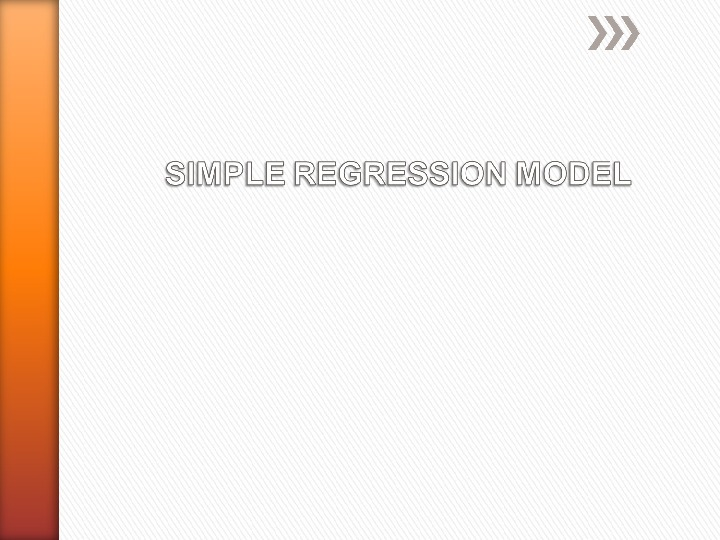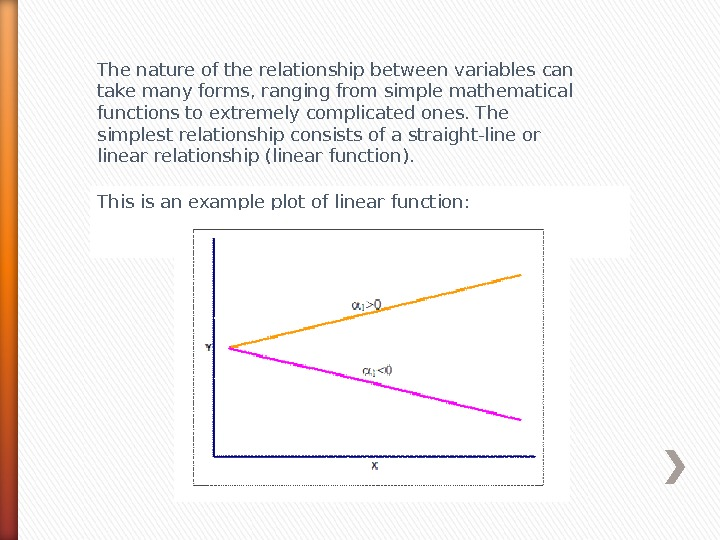This is an example plot of linear function: The nature of the relationship between variables can take many forms, ranging from simple mathematical functions to extremely complicated ones. The simplest relationship consists of a straight-line or linear relationship (linear function).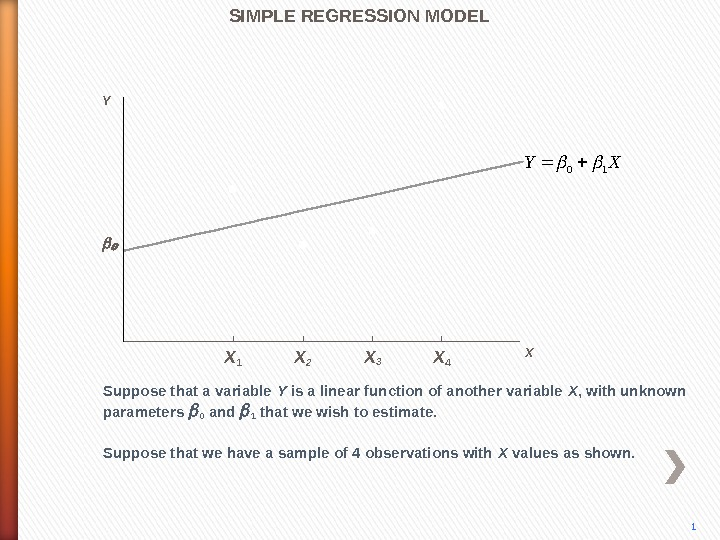1 Y SIMPLE REGRESSION MODEL Suppose that a variable Y is a linear function of another variable X , with unknown parameters 0 and 1 that we wish to estimate. XY 10 X X 1 X 2 X 3 X 4 Suppose that we have a sample of 4 observations with X values as shown.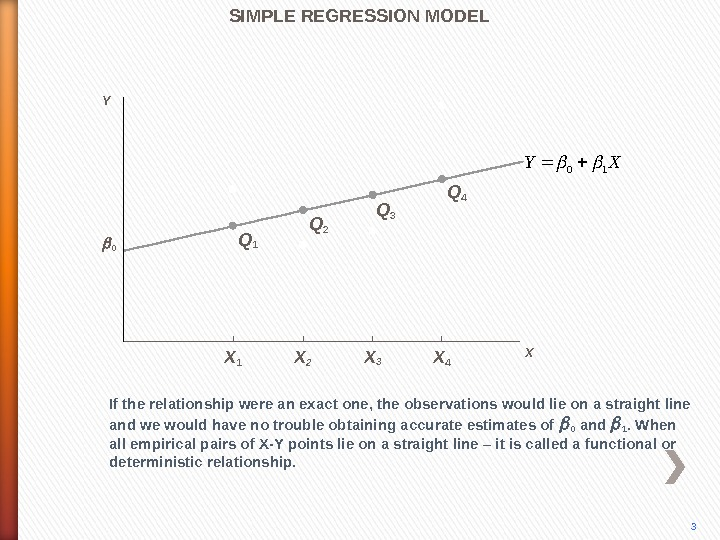If the relationship were an exact one, the observations would lie on a straight line and we would have no trouble obtaining accurate estimates of 0 and 1. When all empirical pairs of X-Y points lie on a straight line – it is called a functional or deterministic relationship. Q 1 Q 2 Q 3 Q 4 SIMPLE REGRESSION MODEL 3 0 Y X X 1 X 2 X 3 X 4 XY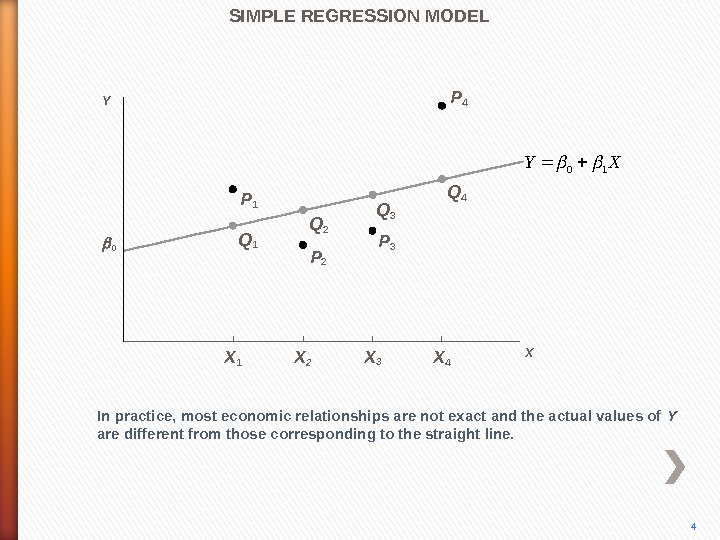P 4 In practice, most economic relationships are not exact and the actual values of Y are different from those corresponding to the straight line. P 3 P 2 P 1 Q 2 Q 3 Q 4 SIMPLE REGRESSION MODEL 4 0 Y X X 1 X 2 X 3 X 4 XY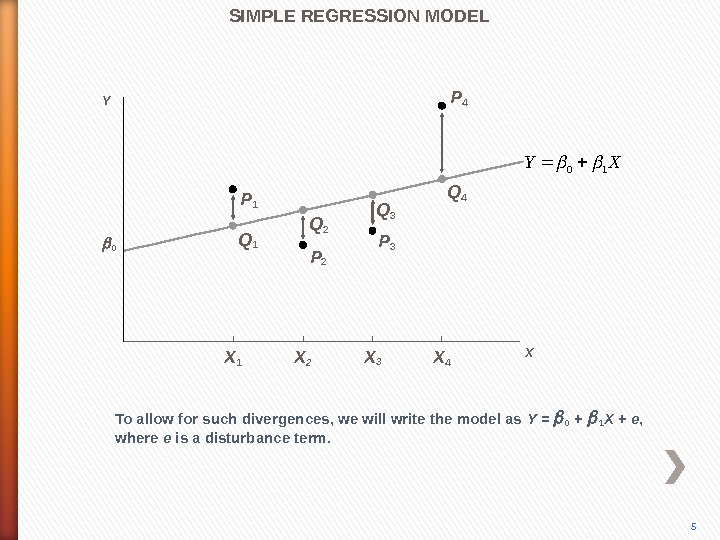P 4 To allow for such divergences, we will write the model as Y = 0 + 1 X + e , where e is a disturbance term. P 3 P 2 P 1 Q 2 Q 3 Q 4 SIMPLE REGRESSION MODEL 5 0 Y X X 1 X 2 X 3 X 4 XY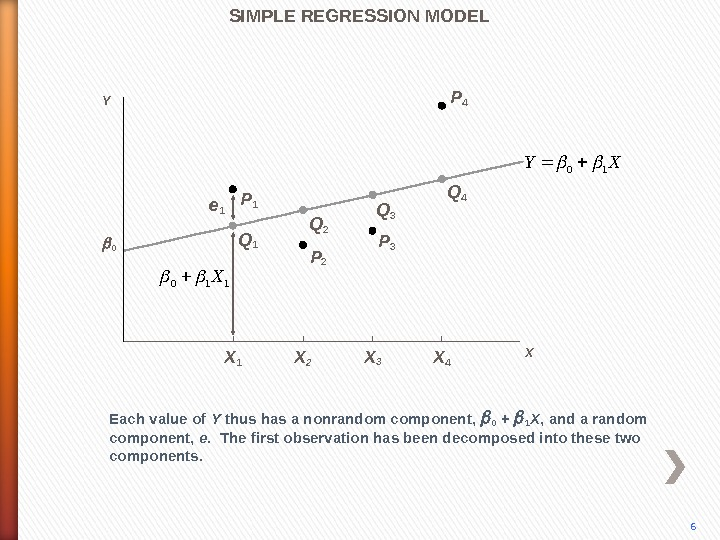P 4 Each value of Y thus has a nonrandom component, 0 + 1 X , and a random component, e. The first observation has been decomposed into these two components. P 3 P 2 P 1 Q 2 Q 3 Q 4 e 1 SIMPLE REGRESSION MODEL 6 0 Y 110 X X X 1 X 2 X 3 X 4 XY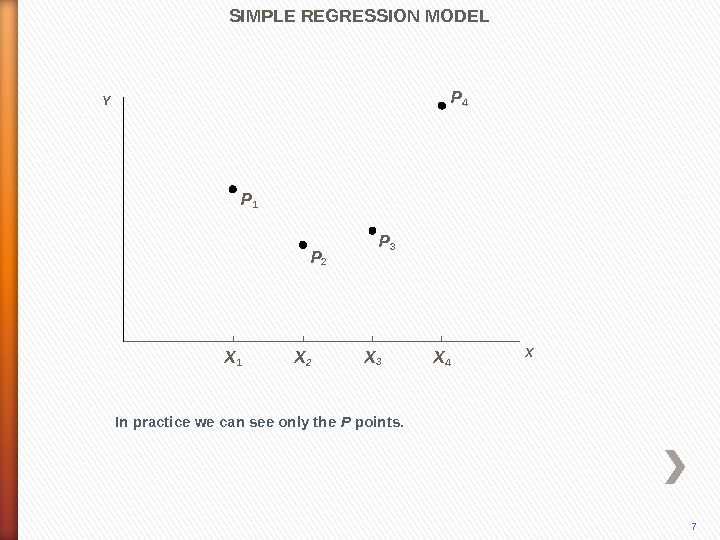P 4 In practice we can see only the P points. P 3 P 2 P 1 SIMPLE REGRESSION MODEL 7 Y X X 1 X 2 X 3 X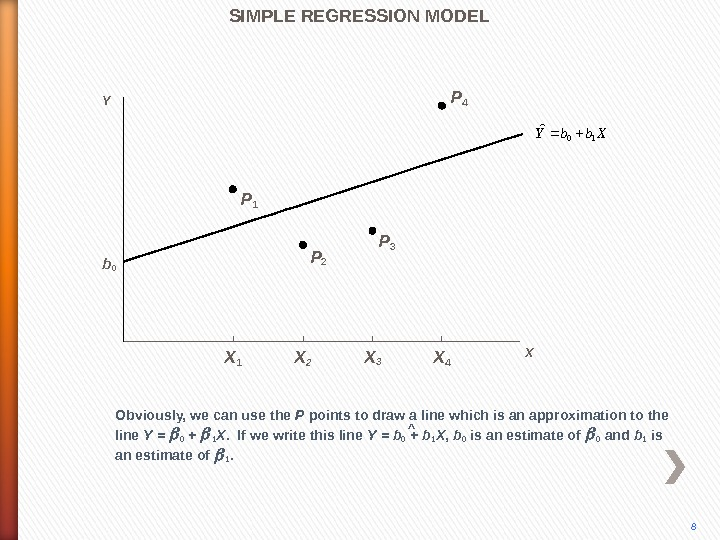P 4 Obviously, we can use the P points to draw a line which is an approximation to the line Y = 0 + 1 X. If we write this line Y = b 0 + b 1 X , b 0 is an estimate of 0 and b 1 is an estimate of 1. P 3 P 2 P 1 ^SIMPLE REGRESSION MODEL 8 Xbb. Y 10ˆb 0 Y X X 1 X 2 X 3 X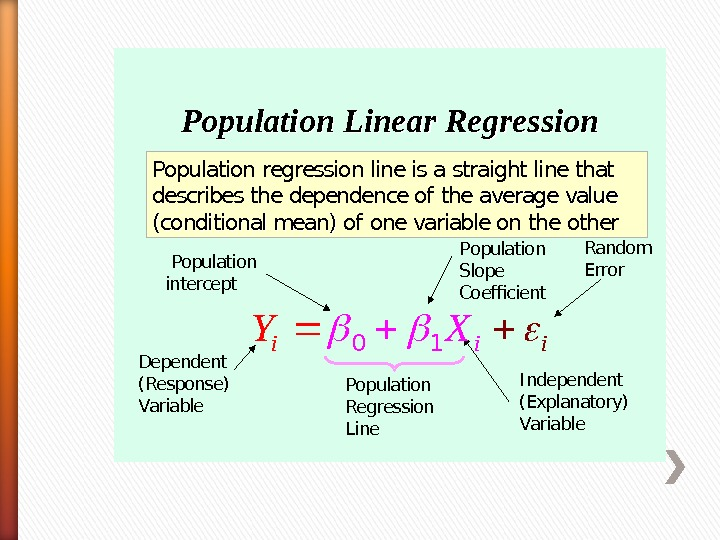Population Regression Line. Population Linear Regression Population regression line is a straight line that describes the dependence of the average value (conditional mean) of one variable on the other Population intercept Population Slope Coefficient Random Error Dependent (Response) Variable I ndependent (Explanatory) Variable iii. YX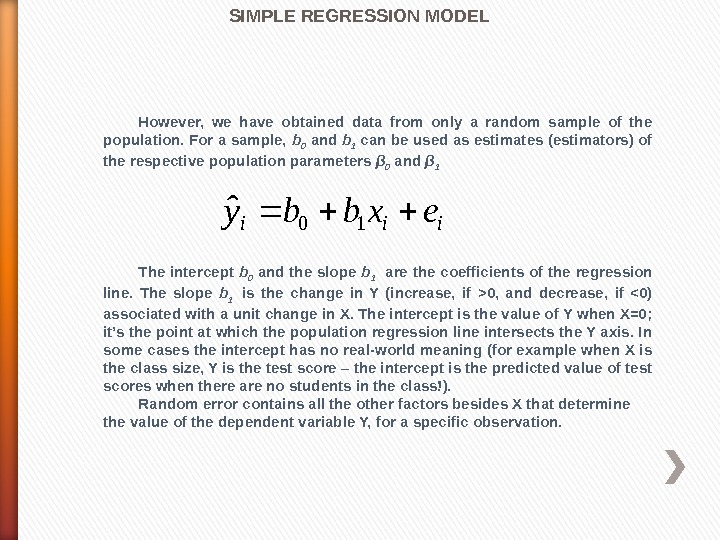However, we have obtained data from only a random sample of the population. For a sample, b 0 and b 1 can be used as estimates (estimators) of the respective population parameters β 0 and β 1 The intercept b 0 and the slope b 1 are the coefficients of the regression line. The slope b 1 is the change in Y (increase, if >0, and decrease, if <0) associated with a unit change in X. The intercept is the value of Y when X=0; it’s the point at which the population regression line intersects the Y axis. In some cases the intercept has no real-world meaning (for example when X is the class size, Y is the test score – the intercept is the predicted value of test scores when there are no students in the class!). Random error contains all the other factors besides X that determine the value of the dependent variable Y, for a specific observation. iiiexbby 10 ˆ SIMPLE REGRESSION MODEL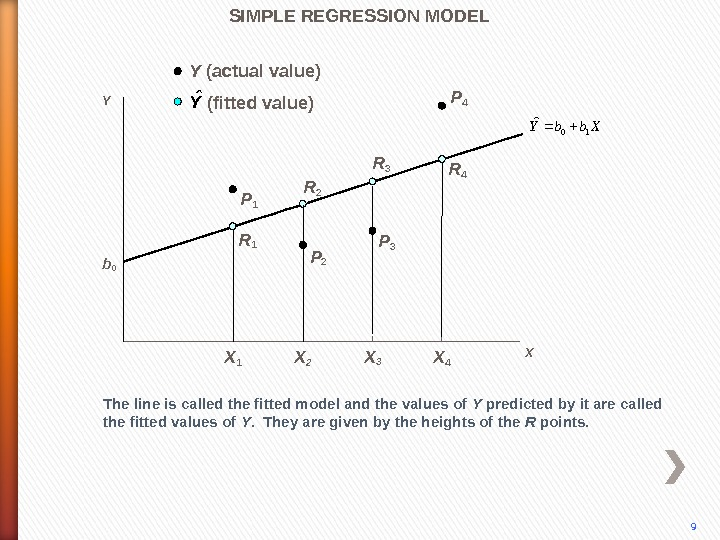P 4 The line is called the fitted model and the values of Y predicted by it are called the fitted values of Y. They are given by the heights of the R points. P 3 P 2 P 1 R 2 R 3 R 4 SIMPLE REGRESSION MODEL 9 b 0 Yˆ (fitted value)Y (actual value) Y X X 1 X 2 X 3 X 4 Xbb. Y 10ˆ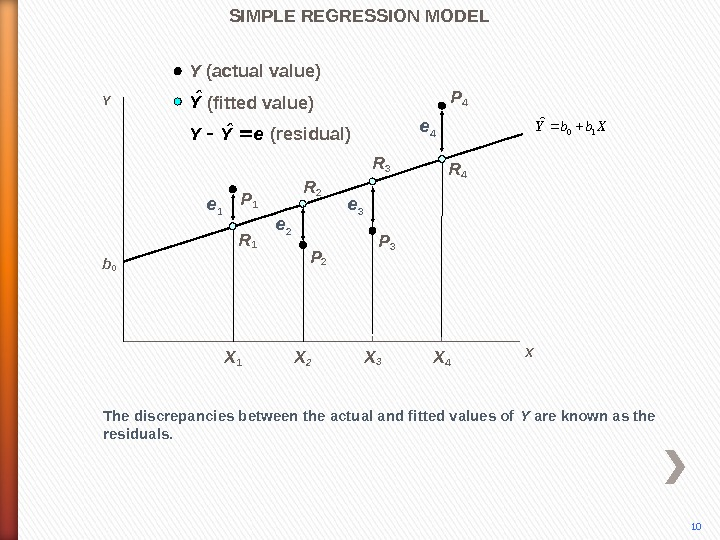P 4 X X 1 X 2 X 3 X 4 The discrepancies between the actual and fitted values of Y are known as the residuals. P 3 P 2 P 1 R 2 R 3 R 4(residual) e 1 e 2 e 3 e 4 SIMPLE REGRESSION MODEL 10 b 0 Yˆ (fitted value)Y (actual value) e. YYˆ YXbb. Y 10ˆ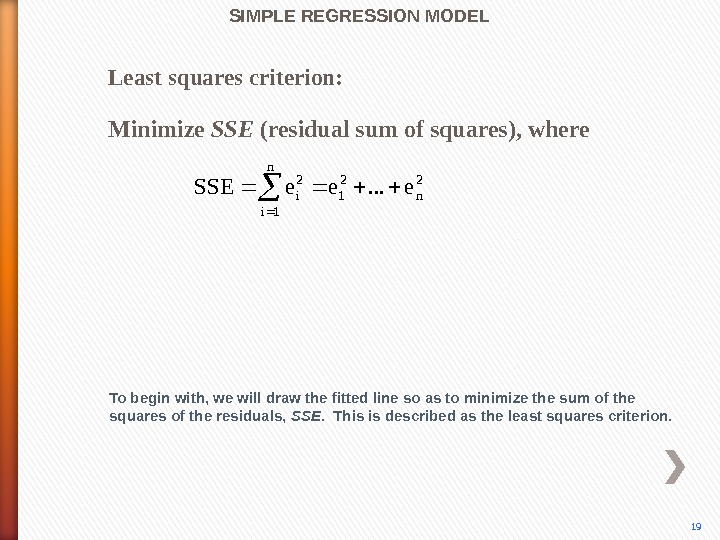SIMPLE REGRESSION MODEL Least squares criterion: 2 n 2 1 n 1 i 2 ie. . . ee. SSE Minimize SSE (residual sum of squares), where To begin with, we will draw the fitted line so as to minimize the sum of the squares of the residuals, SSE. This is described as the least squares criterion.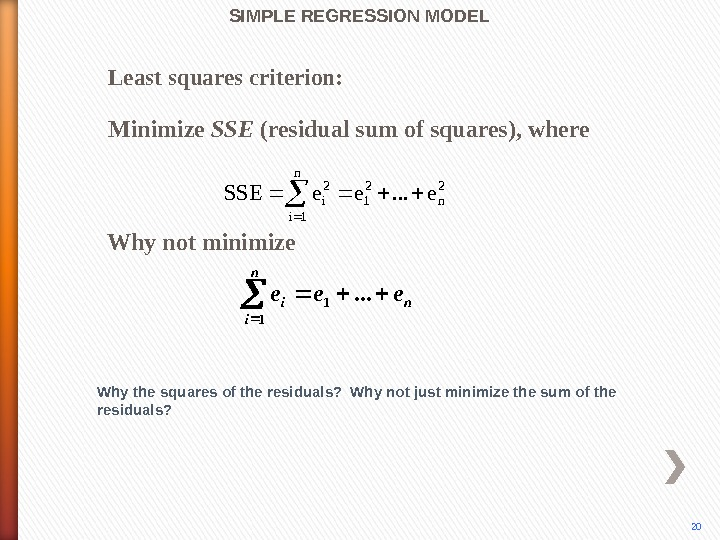SIMPLE REGRESSION MODEL Why the squares of the residuals? Why not just minimize the sum of the residuals? Least squares criterion: Why not minimize 2 n 2 1 n 1 i 2 ie. . . ee. SSΕ nn i i eee . . . 1 1 20 Minimize SSE (residual sum of squares), where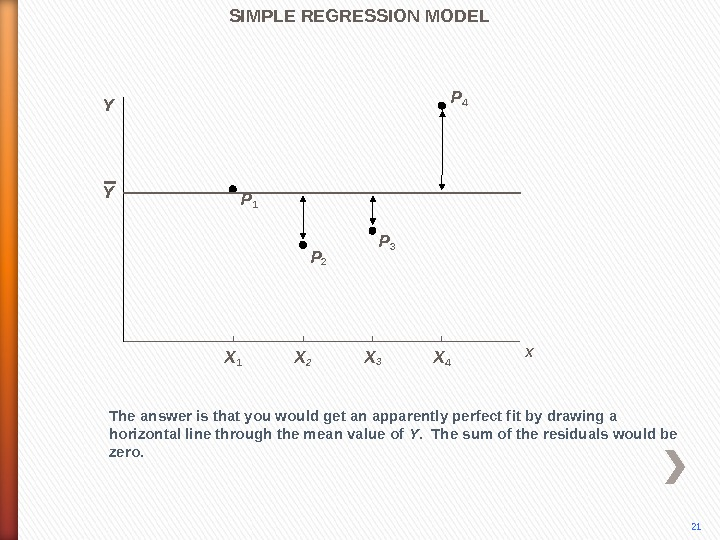P 4 The answer is that you would get an apparently perfect fit by drawing a horizontal line through the mean value of Y. The sum of the residuals would be zero. P 3 P 2 P 1 SIMPLE REGRESSION MODEL Y 21 X X 1 X 2 X 3 X 4 Y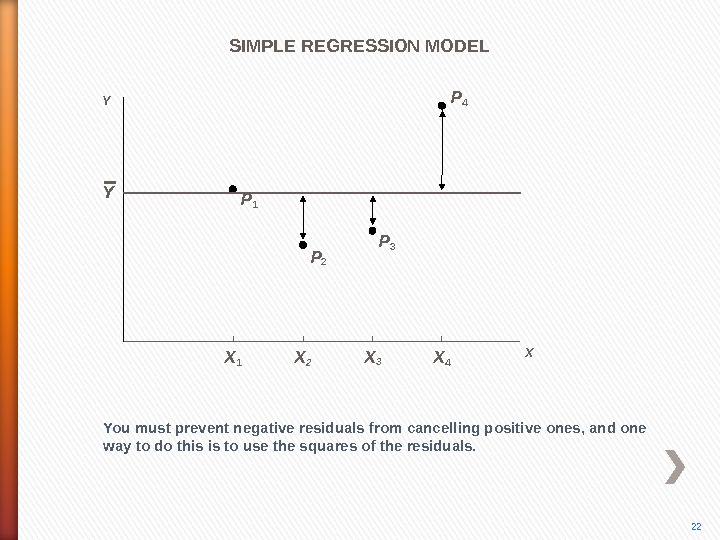P 4 You must prevent negative residuals from cancelling positive ones, and one way to do this is to use the squares of the residuals. P 3 P 2 P 1 SIMPLE REGRESSION MODEL 22 X X 1 X 2 X 3 X 4 Y Y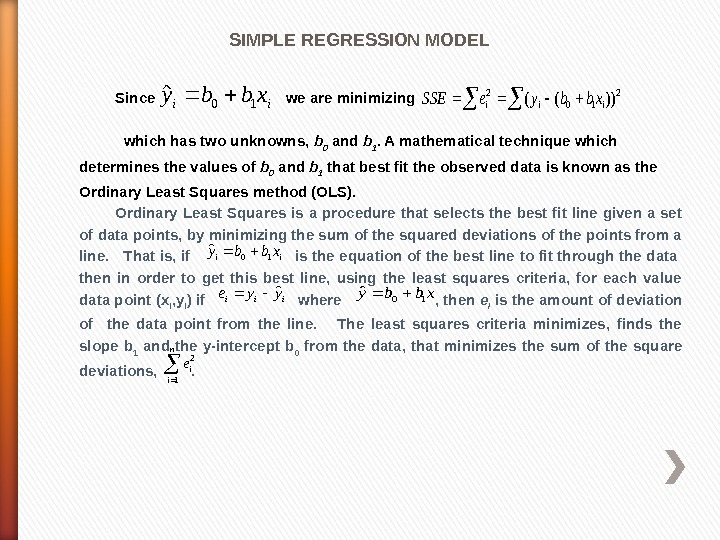SIMPLE REGRESSION MODEL Since we are minimizing which has two unknowns, b 0 and b 1. A mathematical technique which determines the values of b 0 and b 1 that best fit the observed data is known as the Ordinary Least Squares method (OLS). Ordinary Least Squares is a procedure that selects the best fit line given a set of data points, by minimizing the sum of the squared deviations of the points from a line. That is, if is the equation of the best line to fit through the data then in order to get this best line, using the least squares criteria, for each value data point ( x i , y i ) if where , then e i is the amount of deviation of the data point from the line. The least squares criteria minimizes, finds the slope b 1 and the y-intercept b 0 from the data, that minimizes the sum of the square deviations, . iixbby 10ˆ2 10 2))((iiixbbye. SSE xbby 10ˆ iii yye ˆ iixbby 10ˆ n i ie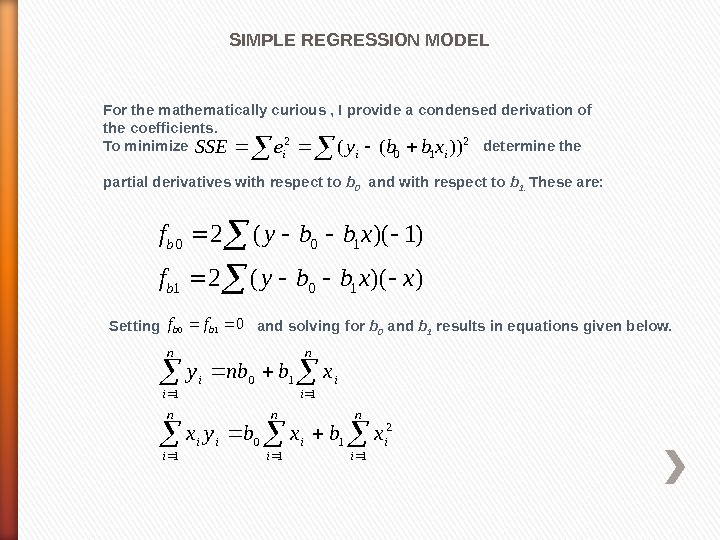SIMPLE REGRESSION MODEL For the mathematically curious , I provide a condensed derivation of the coefficients. To minimize determine the partial derivatives with respect to b 0 and with respect to b 1. These are: 2 10 2))((iiixbbye. SSE ))((2 )1)((2 101 100 xxbbyf bb Setting and solving for b 0 and b 1 results in equations given below. 010 bbff n i iii n i ii xbxbyx xbnby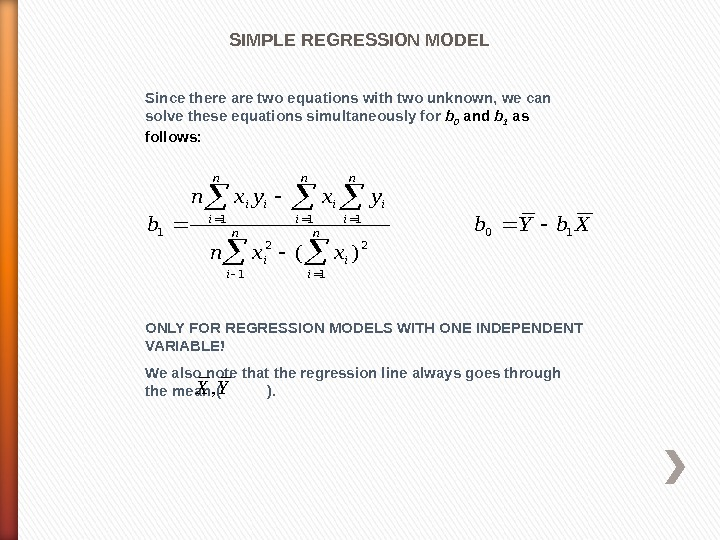Since there are two equations with two unknown, we can solve these equations simultaneously for b 0 and b 1 as follows: ONLY FOR REGRESSION MODELS WITH ONE INDEPENDENT VARIABLE! We also note that the regression line always goes through the mean ( ). Xb. Yb xxn yxyxn bn i i n i iii 10 1 2 111 1 )( , X YSIMPLE REGRESSION MODEL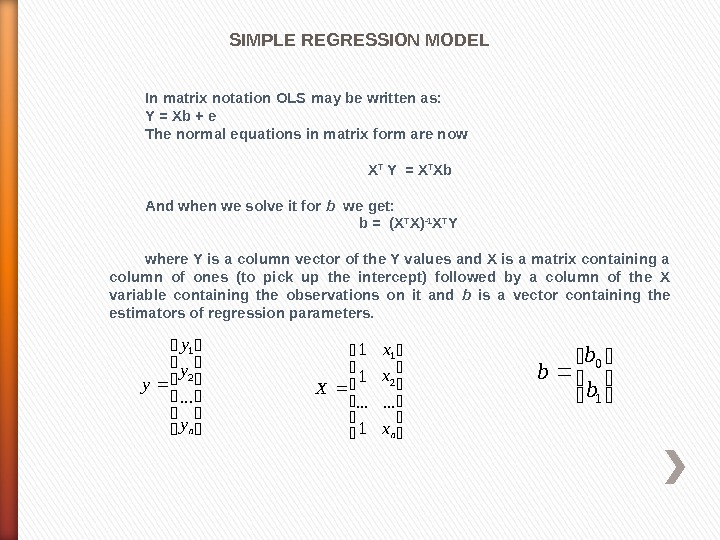SIMPLE REGRESSION MODEL In matrix notation OLS may be written as: Y = Xb + e The normal equations in matrix form are now X T Y = X T Xb And when we solve it for b we get: b = (X T X) -1 X T Y where Y is a column vector of the Y values and X is a matrix containing a column of ones (to pick up the intercept) followed by a column of the X variable containing the observations on it and b is a vector containing the estimators of regression parameters. ny y y y. . . 2 1 nx x x X. . . 1 1 2 1 1 0 b b b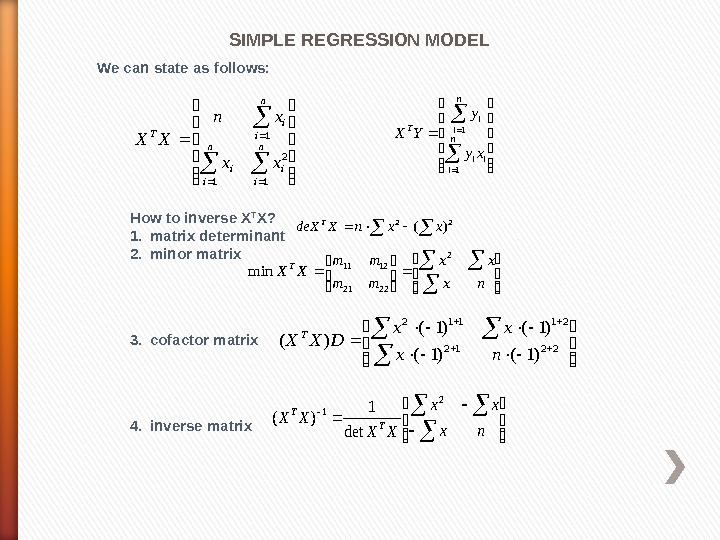SIMPLE REGRESSION MODEL We can state as follows: n i i T xx xn XX 1 2 1 1 n i ii n i i. T xy y YX 1 1 How to inverse X T X? 1. matrix determinant 2. minor matrix 3. cofactor matrix 4. inverse matrix 22 )( xxn. Xde. XT nx xx mm mm XXT 2 2221 1211 min 2212 21112 )1()1( )( nx xx DXXT nx xx XX XXT T 2 1 det 1 )(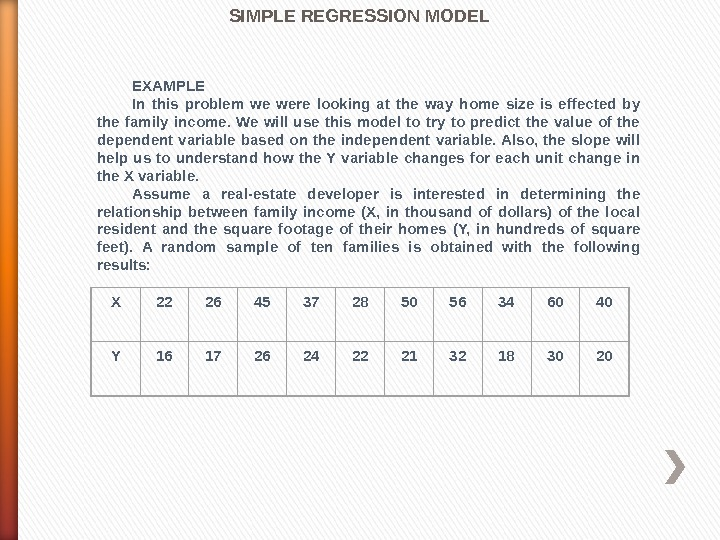SIMPLE REGRESSION MODEL EXAMPLE In this problem we were looking at the way home size is effected by the family income. We will use this model to try to predict the value of the dependent variable based on the independent variable. Also, the slope will help us to understand how the Y variable changes for each unit change in the X variable. Assume a real-estate developer is interested in determining the relationship between family income (X, in thousand of dollars) of the local resident and the square footage of their homes (Y, in hundreds of square feet). A random sample of ten families is obtained with the following results: X 22 26 45 37 28 50 56 34 60 4 0 Y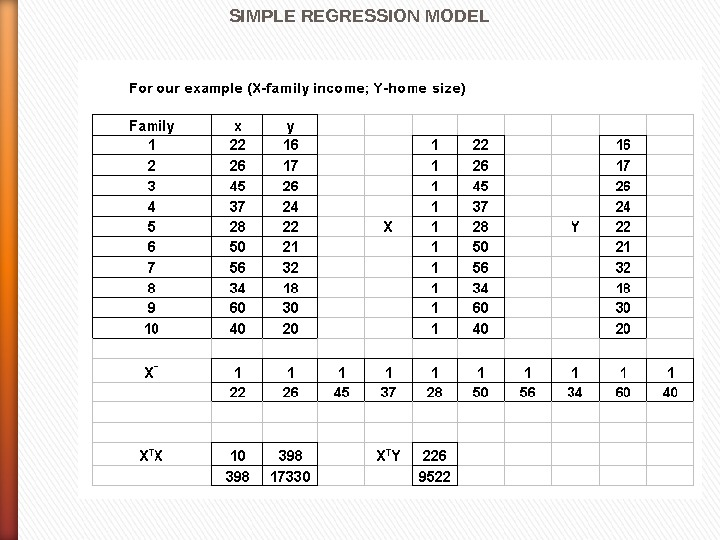SIMPLE REGRESSION MODEL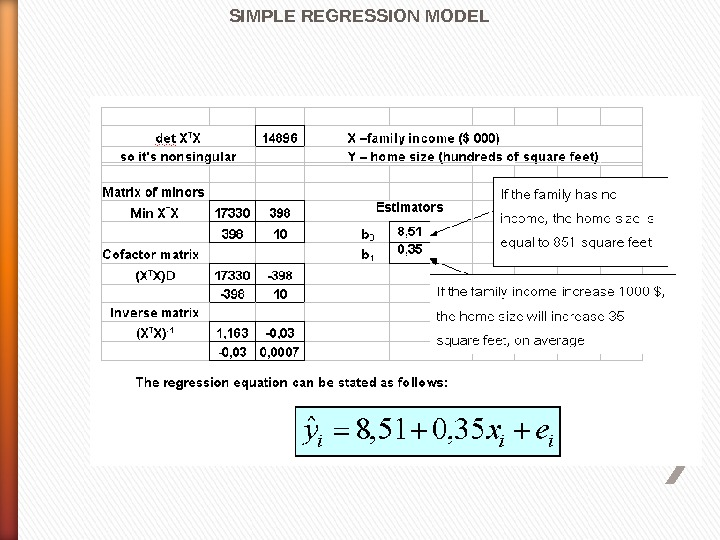SIMPLE REGRESSION MODEL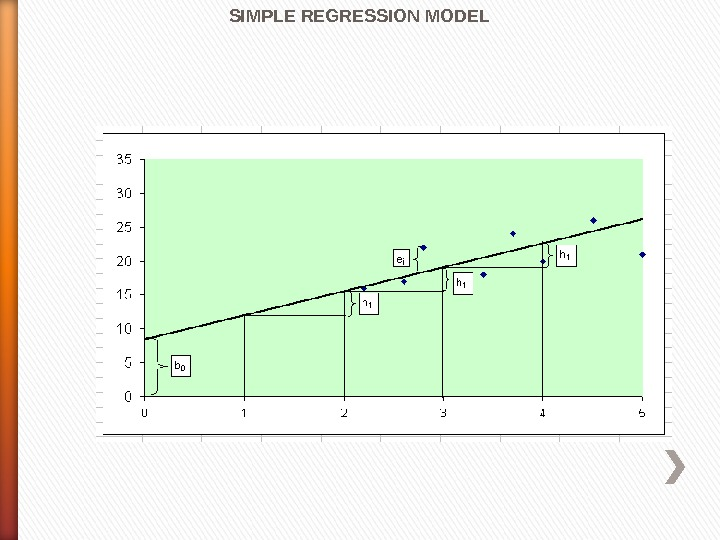SIMPLE REGRESSION MODEL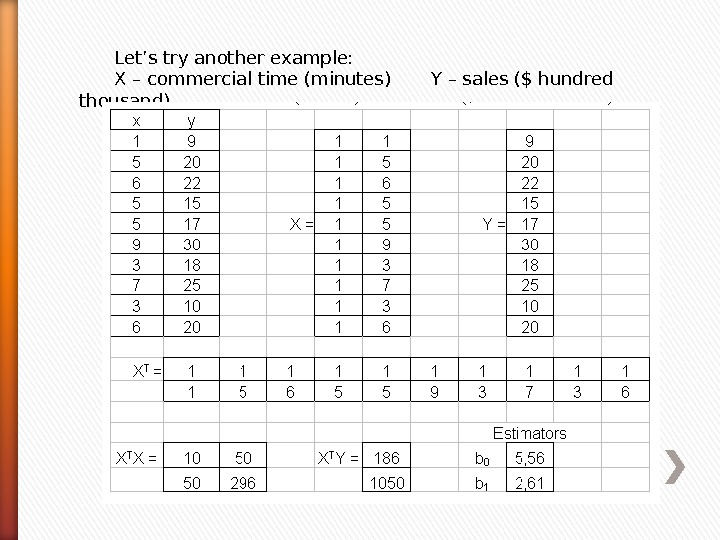Let’s try another example: X – commercial time (minutes) Y – sales (\$ hundred thousand)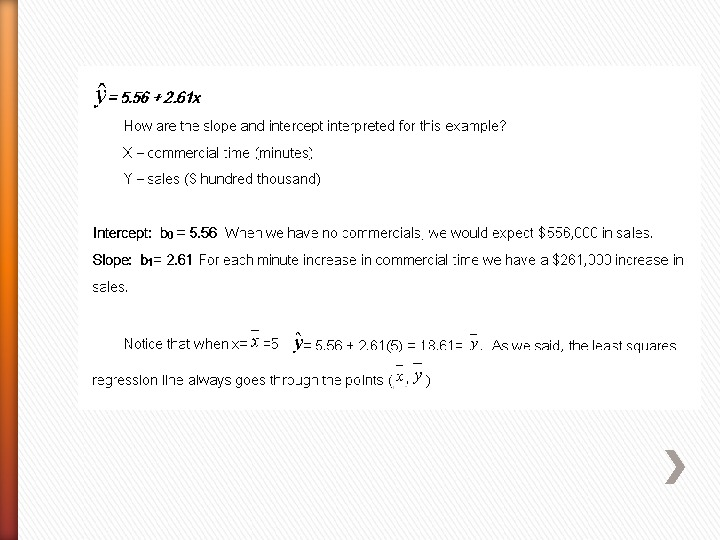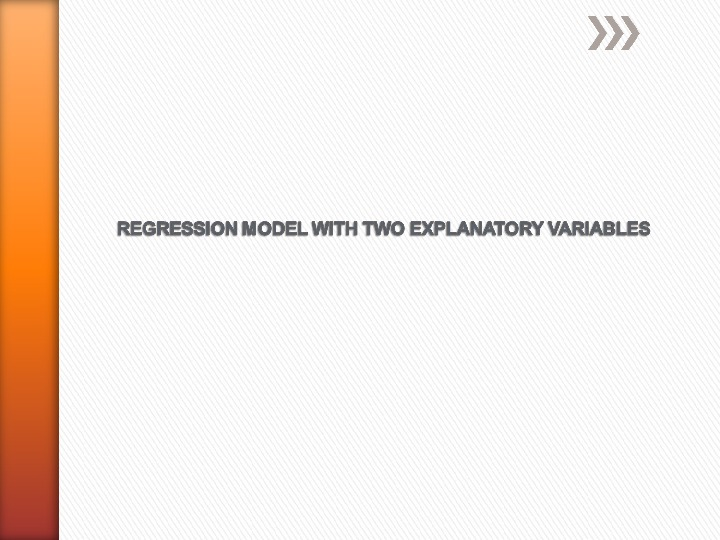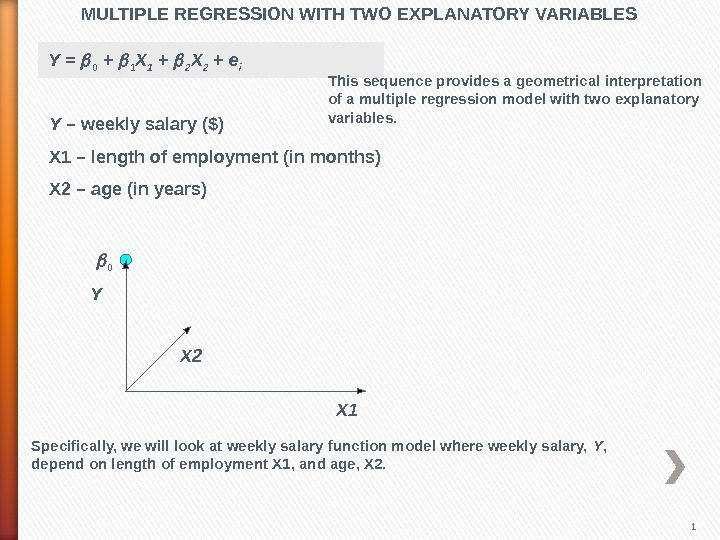MULTIPLE REGRESSION WITH TWO EXPLANATORY VARIABLES Y X 2 X 10 Y = 0 + 1 X 1 + 2 X 2 + e i 1 This sequence provides a geometrical interpretation of a multiple regression model with two explanatory variables. Y – weekly salary (\$) X 1 – length of employment (in months) X 2 – age (in years) Specifically, we will look at weekly salary function model where weekly salary, Y , depend on length of employment X 1, and age, X 2.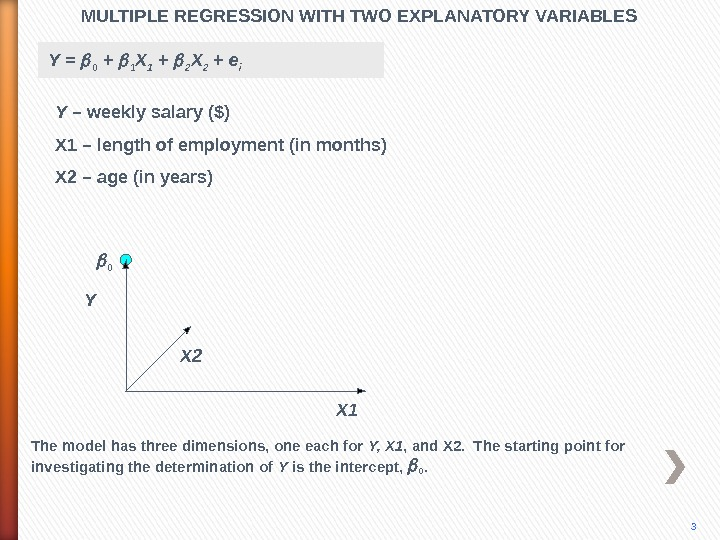MULTIPLE REGRESSION WITH TWO EXPLANATORY VARIABLES Y X 2 X 10 3 The model has three dimensions, one each for Y, X 1 , and X 2. The starting point for investigating the determination of Y is the intercept, 0. Y = 0 + 1 X 1 + 2 X 2 + e i Y – weekly salary (\$) X 1 – length of employment (in months) X 2 – age (in years)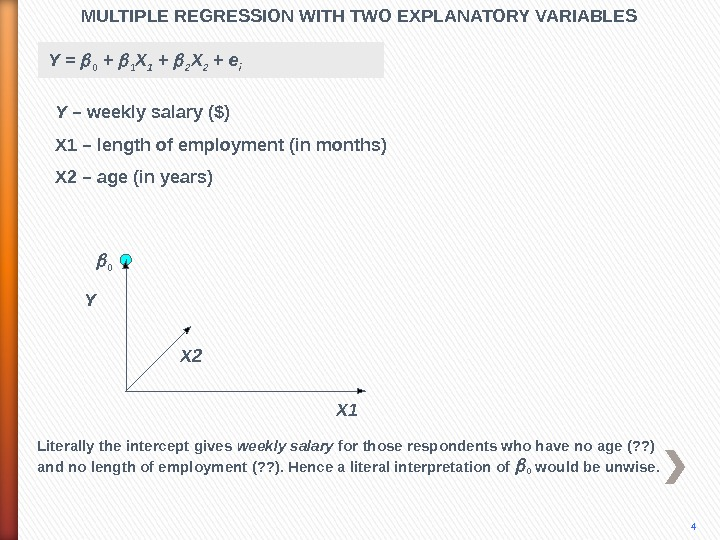MULTIPLE REGRESSION WITH TWO EXPLANATORY VARIABLES Y X 2 X 10 4 Literally the intercept gives weekly salary for those respondents who have no age (? ? ) and no length of employment (? ? ). Hence a literal interpretation of 0 would be unwise. Y = 0 + 1 X 1 + 2 X 2 + e i Y – weekly salary (\$) X 1 – length of employment (in months) X 2 – age (in years)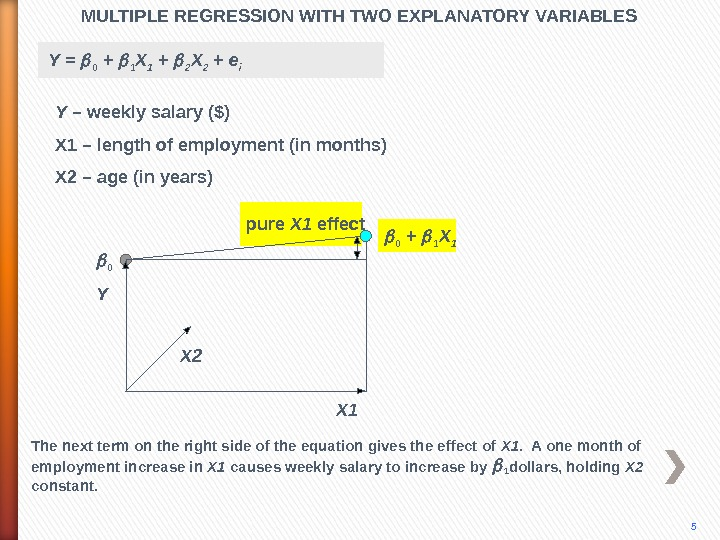MULTIPLE REGRESSION WITH TWO EXPLANATORY VARIABLES 5 Y X 2 The next term on the right side of the equation gives the effect of X 1. A one month of employment increase in X 1 causes weekly salary to increase by 1 dollars, holding X 2 constant. X 1 0 pure X 1 effect 0 + 1 X 1 Y = 0 + 1 X 1 + 2 X 2 + e i Y – weekly salary (\$) X 1 – length of employment (in months) X 2 – age (in years)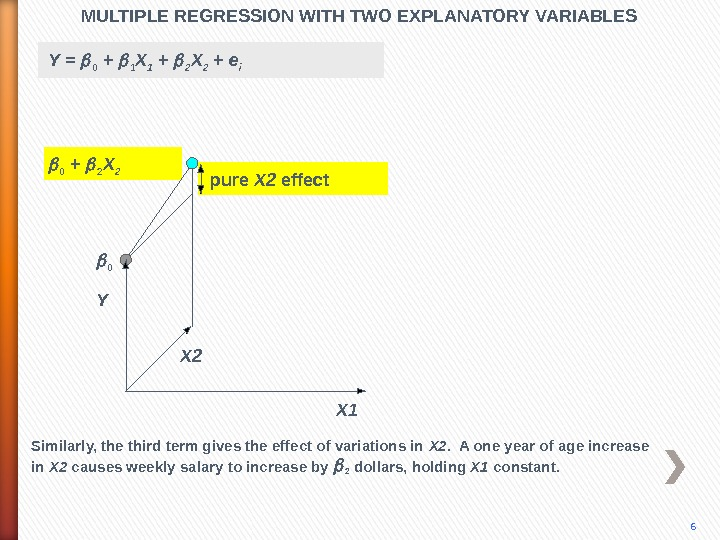pure X 2 effect. MULTIPLE REGRESSION WITH TWO EXPLANATORY VARIABLES X 10 0 + 2 X 2 Y X 2 6 Similarly, the third term gives the effect of variations in X 2. A one year of age increase in X 2 causes weekly salary to increase by 2 dollars, holding X 1 constant. Y = 0 + 1 X 1 + 2 X 2 + e i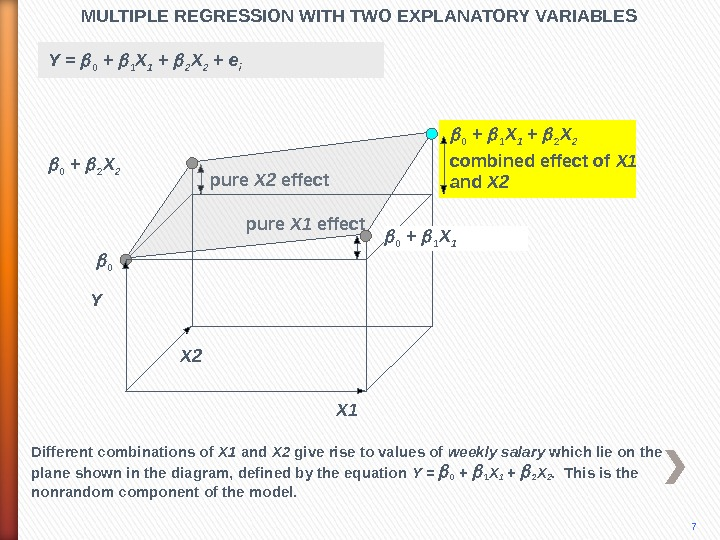pure X 2 effect pure X 1 effect. MULTIPLE REGRESSION WITH TWO EXPLANATORY VARIABLES X 10 0 + 2 X 2 0 + 1 X 1 + 2 X 2 Y X 2 0 + 1 X 1 combined effect of X 1 and X 2 7 Different combinations of X 1 and X 2 give rise to values of weekly salary which lie on the plane shown in the diagram, defined by the equation Y = 0 + 1 X 1 + 2 X 2. This is the nonrandom component of the model. Y = 0 + 1 X 1 + 2 X 2 + e i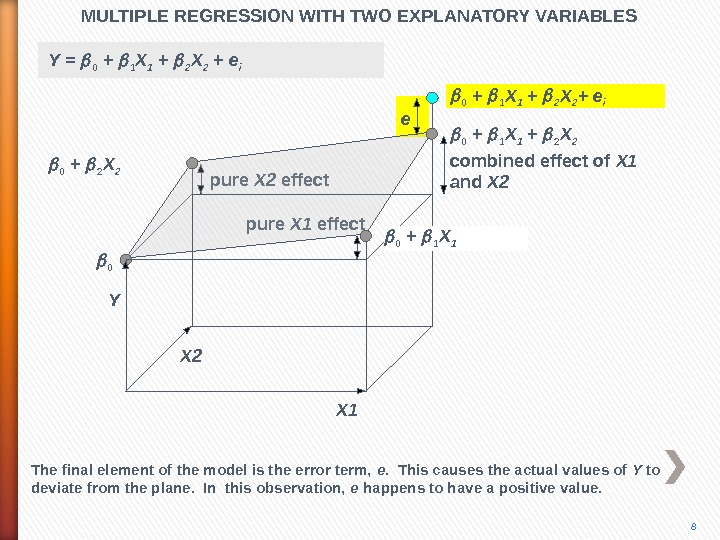pure X 2 effect pure X 1 effect. MULTIPLE REGRESSION WITH TWO EXPLANATORY VARIABLES X 10 0 + 2 X 2 0 + 1 X 1 + 2 X 2 + e i Y X 2 combined effect of X 1 and X 2 e 8 The final element of the model is the error term, e. This causes the actual values of Y to deviate from the plane. In this observation, e happens to have a positive value. 0 + 1 X 1 Y = 0 + 1 X 1 + 2 X 2 + e i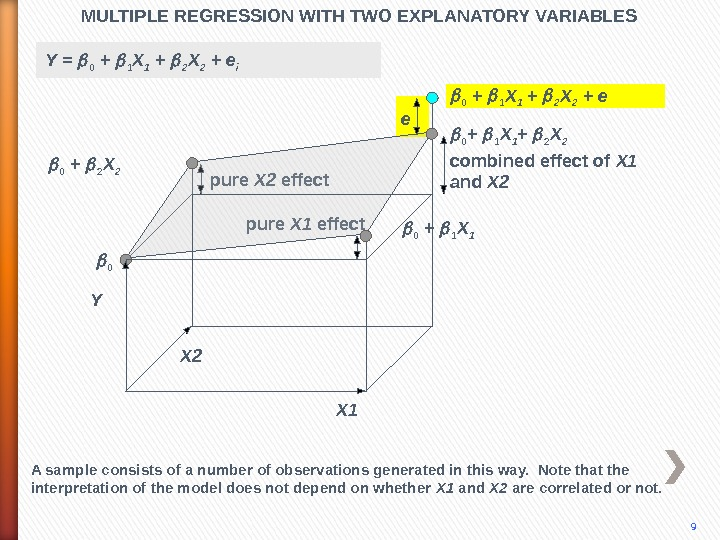pure X 2 effect pure X 1 effect. MULTIPLE REGRESSION WITH TWO EXPLANATORY VARIABLES X 10 0 + 1 X 1 + 2 X 2 0 + 1 X 1 + 2 X 2 + e Y X 2 combined effect of X 1 and X 2 e 9 A sample consists of a number of observations generated in this way. Note that the interpretation of the model does not depend on whether X 1 and X 2 are correlated or not. 0 + 1 X 1 Y = 0 + 1 X 1 + 2 X 2 + e i 0 + 2 X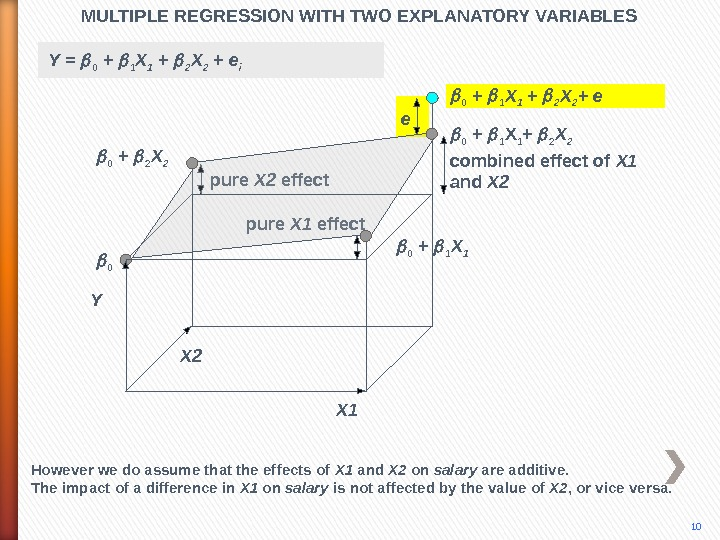pure X 2 effect pure X 1 effect. MULTIPLE REGRESSION WITH TWO EXPLANATORY VARIABLES 10 X 10 0 + 1 X 1 + 2 X 2 + e Y X 2 combined effect of X 1 and X 2 e However we do assume that the effects of X 1 and X 2 on salary are additive. The impact of a difference in X 1 on salary is not affected by the value of X 2 , or vice versa. 0 + 1 X 1 Y = 0 + 1 X 1 + 2 X 2 + e i 0 + 2 X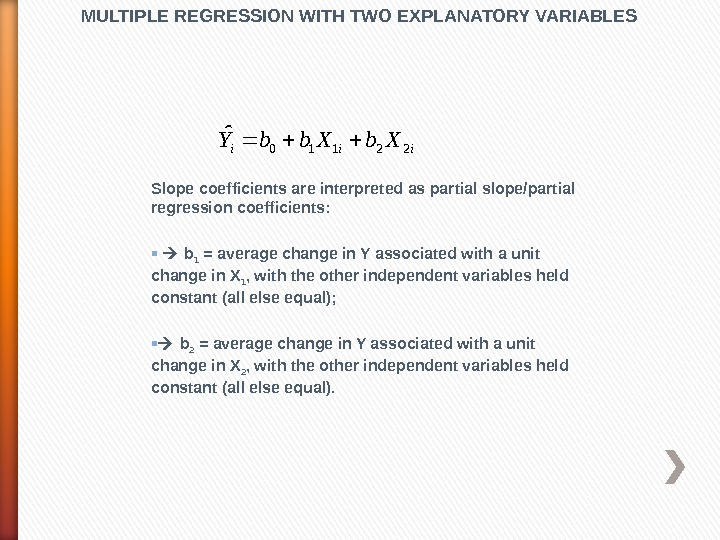Slope coefficients are interpreted as partial slope/partial regression coefficients: b 1 = average change in Y associated with a unit change in X 1 , with the other independent variables held constant (all else equal); b 2 = average change in Y associated with a unit change in X 2 , with the other independent variables held constant (all else equal). MULTIPLE REGRESSION WITH TWO EXPLANATORY VARIABLESiii. Xbb. Y 22110 ˆ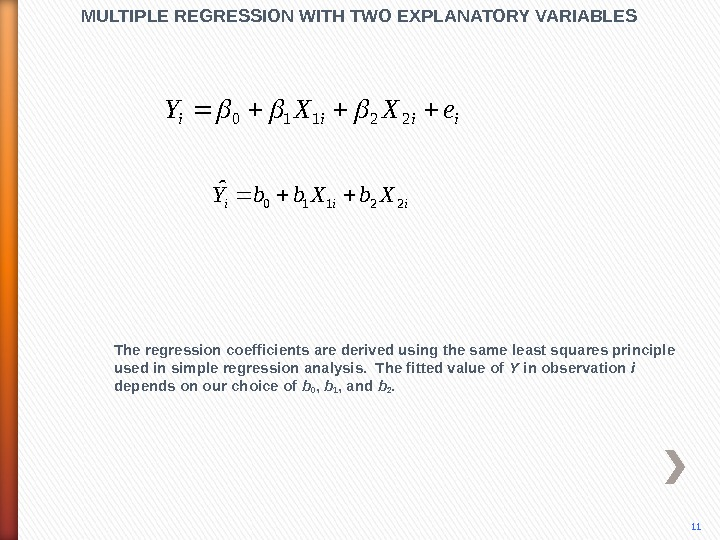iiii e. XXY 22110 iii. Xbb. Y 22110 ˆMULTIPLE REGRESSION WITH TWO EXPLANATORY VARIABLES The regression coefficients are derived using the same least squares principle used in simple regression analysis. The fitted value of Y in observation i depends on our choice of b 0 , b 1 , and b 2.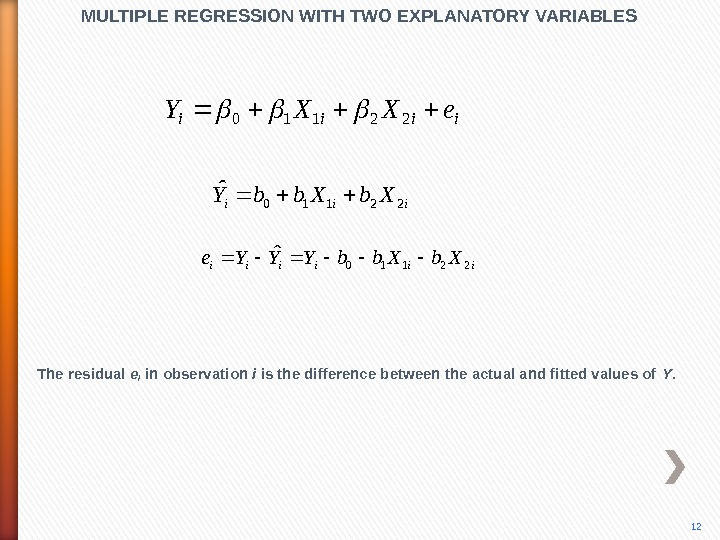iiiiii. Xbb. YYYe 22110 ˆMULTIPLE REGRESSION WITH TWO EXPLANATORY VARIABLES The residual e i in observation i is the difference between the actual and fitted values of Y. 12 iiii e. XXY 22110 iii. Xbb. Y 22110 ˆ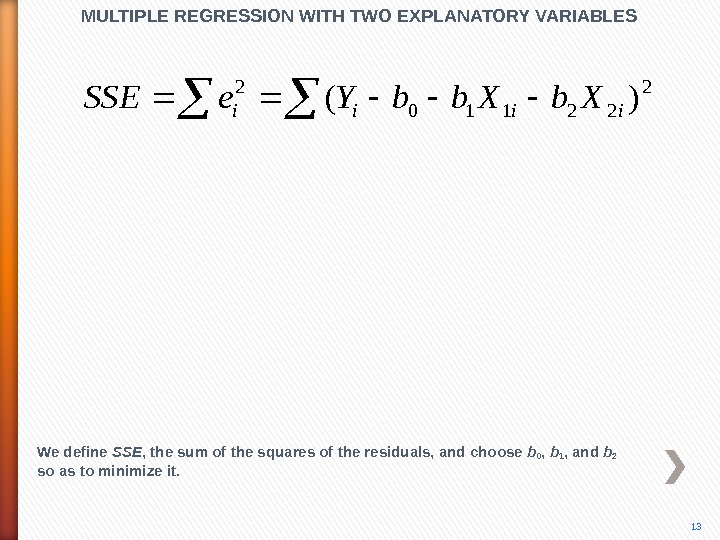2 22110 2 )(iiii. Xbb. Ye. SSEMULTIPLE REGRESSION WITH TWO EXPLANATORY VARIABLES We define SSE , the sum of the squares of the residuals, and choose b 0 , b 1 , and b 2 so as to minimize it.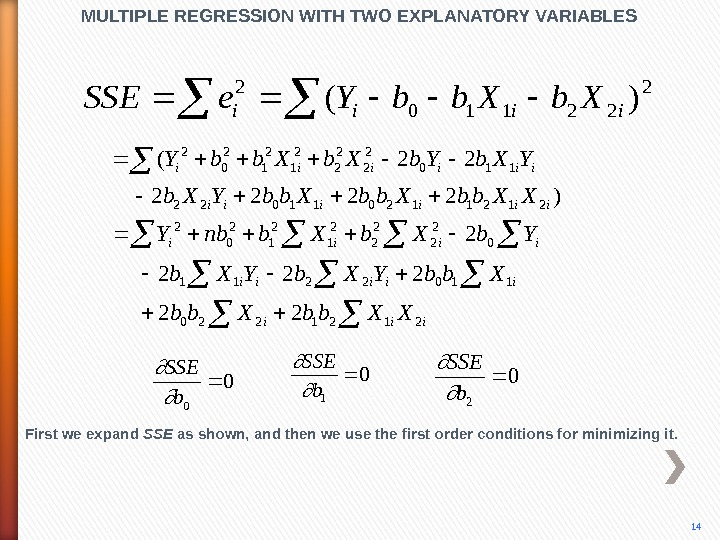)2222 22( 212112011022 110 2 2 2 1 2 0 2 iiiiii XXbb. YXb. Yb. Xbb. Y iiiii XXbb. YXb Yb. Xbnb. Y 2121220 1102211 0 2 2 2 1 2 0 2 22 20 0 b. SSE 0 1 b. SSE 0 2 b. SSE MULTIPLE REGRESSION WITH TWO EXPLANATORY VARIABLES First we expand SSE as shown, and then we use the first order conditions for minimizing it. 14 2 22110 2 )(iiii. Xbb. Ye. SS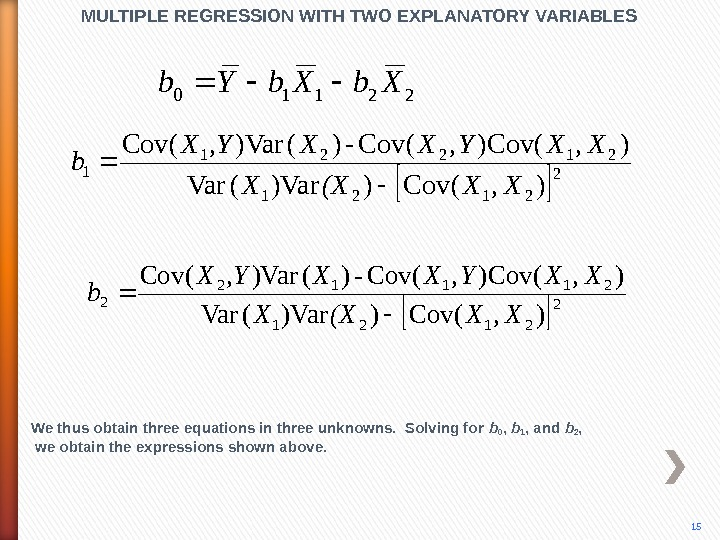22110 Xb. Yb 2 2121 21221 1), (Cov))Var(Var ), (Cov-)()Var(Cov XX(XX XXYXX, YX b MULTIPLE REGRESSION WITH TWO EXPLANATORY VARIABLES We thus obtain three equations in three unknowns. Solving for b 0 , b 1 , and b 2 , we obtain the expressions shown above. 15 2 2121 21112 2 ), (Cov))Var(Var ), (Cov-)()Var(Cov XX(XX XXYXX, YX b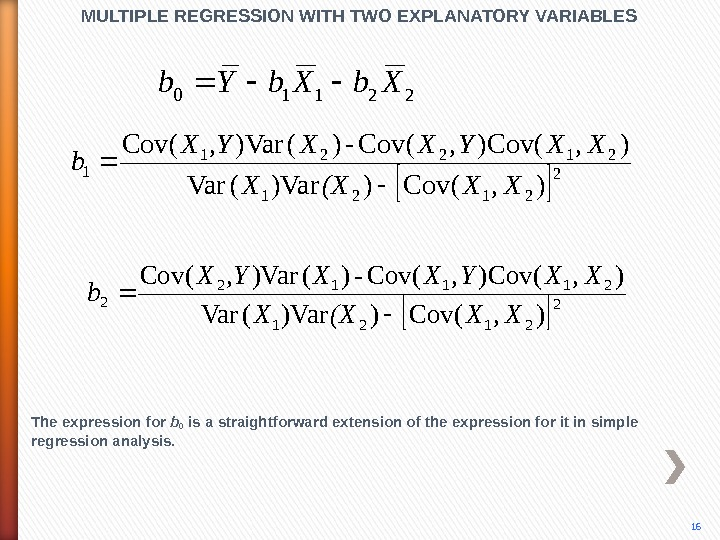MULTIPLE REGRESSION WITH TWO EXPLANATORY VARIABLES The expression for b 0 is a straightforward extension of the expression for it in simple regression analysis. 16 22110 Xb. Yb 2 2121 21221 1), (Cov))Var(Var ), (Cov-)()Var(Cov XX(XX XXYXX, YX b 2 2121 21112 2 ), (Cov))Var(Var ), (Cov-)()Var(Cov XX(XX XXYXX, YX b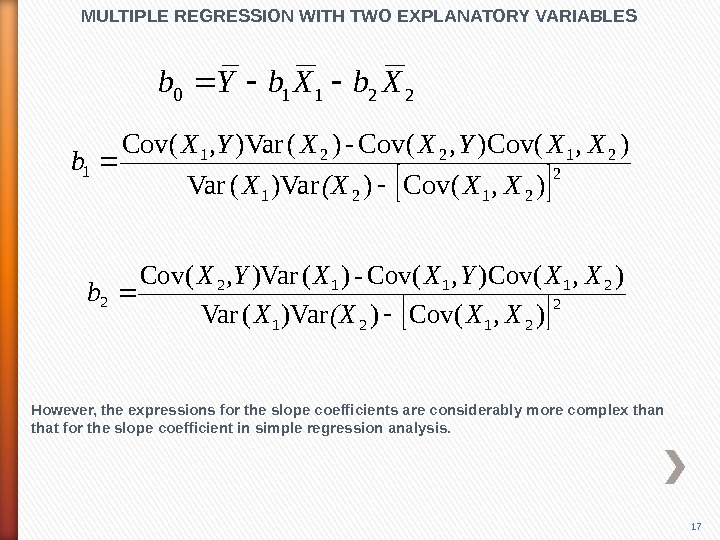MULTIPLE REGRESSION WITH TWO EXPLANATORY VARIABLES However, the expressions for the slope coefficients are considerably more complex than that for the slope coefficient in simple regression analysis. 1722110 Xb. Yb 2 2121 21221 1), (Cov))Var(Var ), (Cov-)()Var(Cov XX(XX XXYXX, YX b 2 2121 21112 2 ), (Cov))Var(Var ), (Cov-)()Var(Cov XX(XX XXYXX, YX b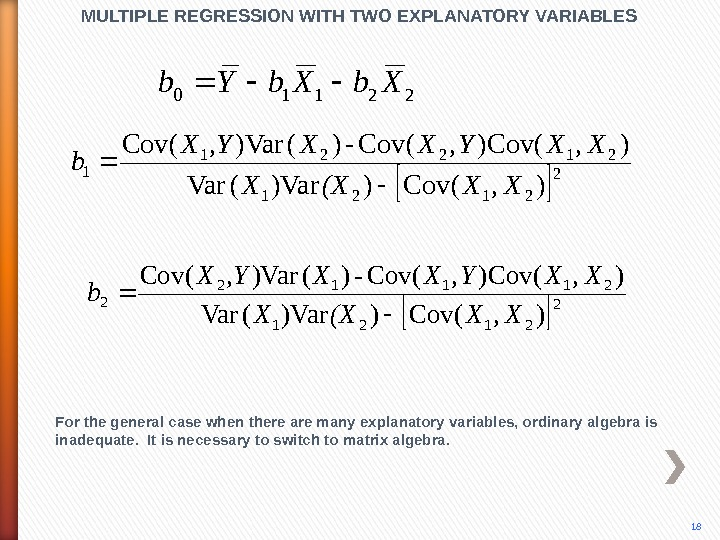MULTIPLE REGRESSION WITH TWO EXPLANATORY VARIABLES For the general case when there are many explanatory variables, ordinary algebra is inadequate. It is necessary to switch to matrix algebra. 1822110 Xb. Yb 2 2121 21221 1), (Cov))Var(Var ), (Cov-)()Var(Cov XX(XX XXYXX, YX b 2 2121 21112 2 ), (Cov))Var(Var ), (Cov-)()Var(Cov XX(XX XXYXX, YX b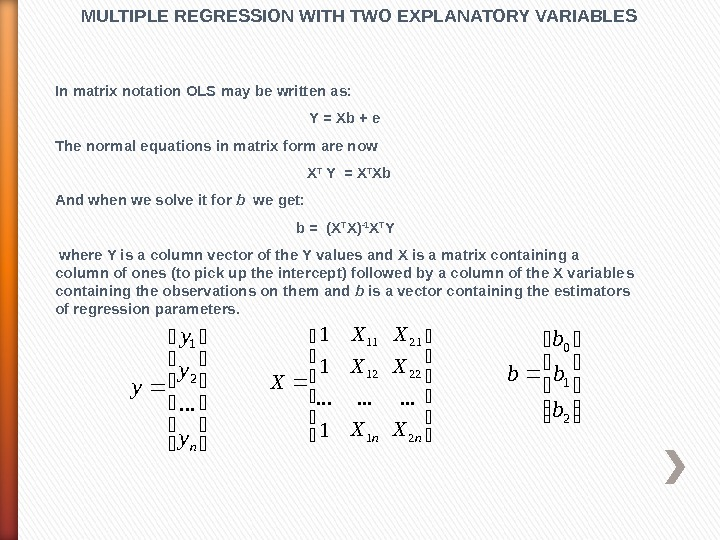In matrix notation OLS may be written as: Y = Xb + e The normal equations in matrix form are now X T Y = X T Xb And when w e solve it for b we get: b = (X T X) -1 X T Y where Y is a column vector of the Y values and X is a matrix containing a column of ones (to pick up the intercept) followed by a column of the X variable s containing the observations on t hem and b is a vector containing the estimators of regression parameters. MULTIPLE REGRESSION WITH TWO EXPLANATORY VARIABLES ny y y y. . . 2 1 21 0 b b nn. X X X X 2 22 21 1 12 11. . .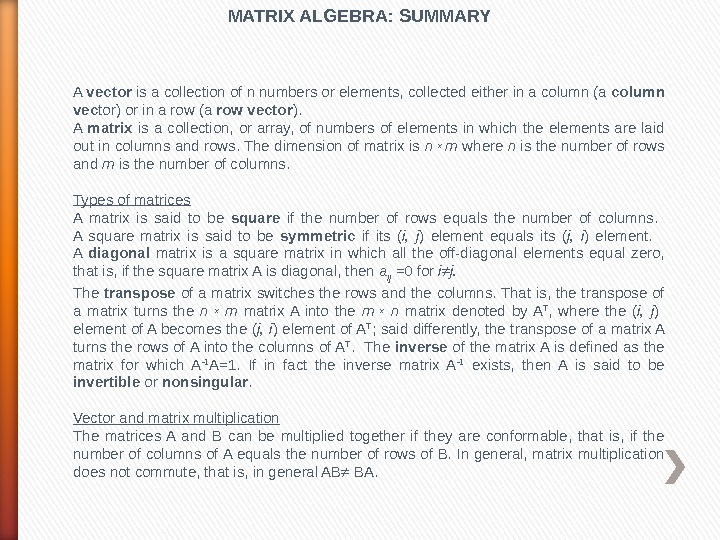MATRIX ALGEBRA: SUMMARY A vector is a collection of n numbers or elements, collected either in a column (a column vec tor) or in a row (a row vector ). A matrix is a collection, or array, of numbers of elements in which the elements are laid out in columns and rows. The dimension of matrix is n x m where n is the number of rows and m is the number of columns. Types of matrices A matrix is said to be square if the number of rows equals the number of columns. A square matrix is said to be symmetric if its ( i, j ) element equals its ( j, i ) element. A diagonal matrix is a square matrix in which all the off-diagonal elements equal zero, that is, if the square matrix A is diagonal, then a ij =0 for i≠j. The transpose of a matrix switches the rows and the columns. That is, the transpose of a matrix turns the n x m matrix A into the m x n matrix denoted by A T , where the ( i, j ) element of A becomes the ( j, i ) element of A T ; said differently, the transpose of a matrix A turns the rows of A into the columns of A T. The inverse of the matrix A is defined as the matrix for which A -1 A= 1. If in fact the inverse matrix A -1 exists, then A is said to be invertible or nonsingular. Vector and matrix multiplication The matrices A and B can be multiplied together if they are conformable, that is, if the number of columns of A equals the number of rows of B. In general, matrix multiplication does not commute, that is, in general AB≠ BA.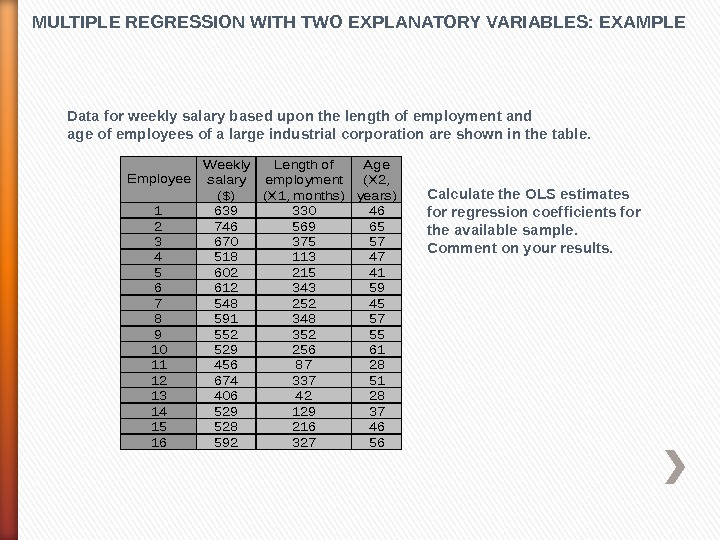MULTIPLE REGRESSION WITH TWO EXPLANATORY VARIABLES: EXAMPLE Data for weekly salary based upon the length of employment and age of employees of a large industrial corporation are shown in the table. 163933046 274656965 367037557 451811347 560221541 661234359 754825245 859134857 955235255 1052925661 114568728 1267433751 134064228 1452912937 1552821646 1659232756 Weekly salary (\$) Length of employment (X 1, months) Age (X 2, years) Employee Calculate the OLS estimates for regression coefficients for the available sample. Comment on your results.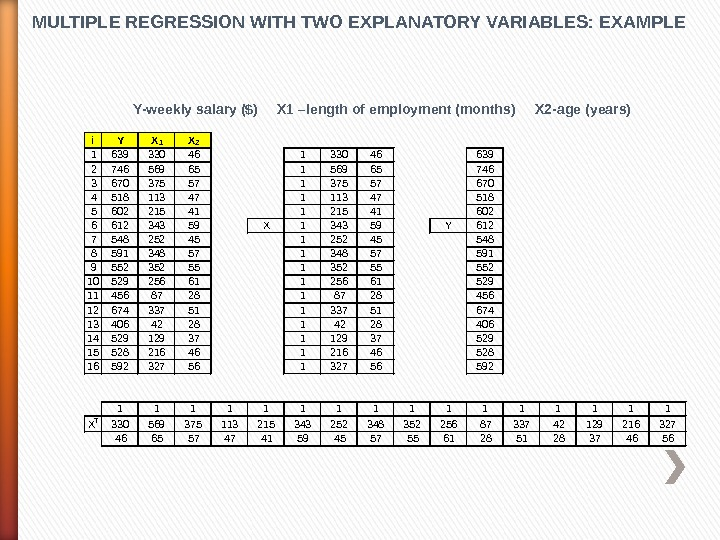MULTIPLE REGRESSION WITH TWO EXPLANATORY VARIABLES: EXAMPLE i Y X 1 X 2 1 639 330 46 1 330 46 639 2 746 569 65 1 569 65 746 3 670 375 57 1 375 57 670 4 518 113 47 1 113 47 518 5 602 215 41 1 215 41 602 6 612 343 59 X 1 343 59 Y 612 7 548 252 45 1 252 45 548 8 591 348 57 591 9 552 352 55 1 352 55 552 10 529 256 61 1 256 61 529 11 456 87 28 1 87 28 456 12 674 337 51 1 337 51 674 13 406 42 28 1 42 28 406 14 529 129 37 1 129 37 529 15 528 216 46 1 216 46 528 16 592 327 56 1 327 56 592 1 1 1 1 X T 330 569 375 113 215 343 252 348 352 256 87 337 42 129 216 327 46 65 57 47 41 59 45 57 55 61 28 51 28 37 46 56 Y-weekly salary (\$) X 1 –length of employment (months) X 2 -age (years)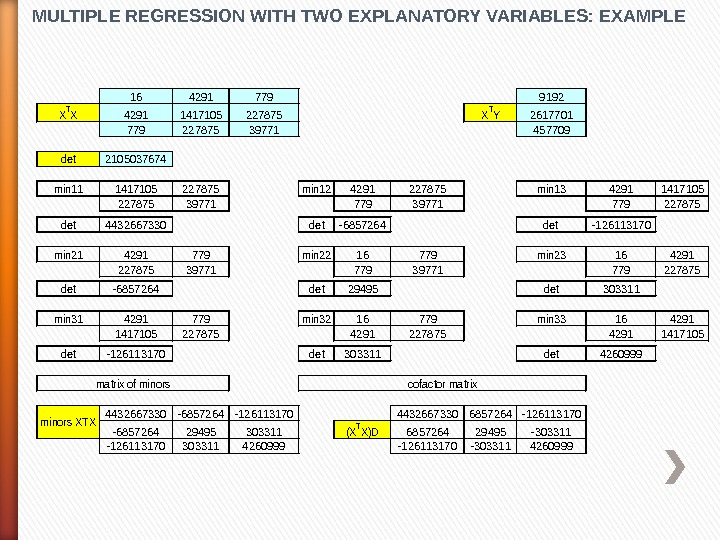MULTIPLE REGRESSION WITH TWO EXPLANATORY VARIABLES: EXAMPLE 1642917799192 XTX 42911417105227875 XTY 2617701 77922787539771457709 det 2105037674 min 111417105227875 min 124291227875 min 1342911417105 22787539771779227875 det 4432667330 det-6857264 det -126113170 min 214291779 min 2216779 min 23164291 22787539771779227875 det-6857264 det 29495 det 303311 min 314291779 min 3216779 min 33164291 141710522787542911417105 det-126113170 det 303311 det 4260999 4432667330 -6857264 -12611317044326673306857264 -126113170 -685726429495303311(XTX)D 685726429495 -303311 -1261131703033114260999 -126113170 -3033114260999 minors XTX matrix of minorscofactor matrix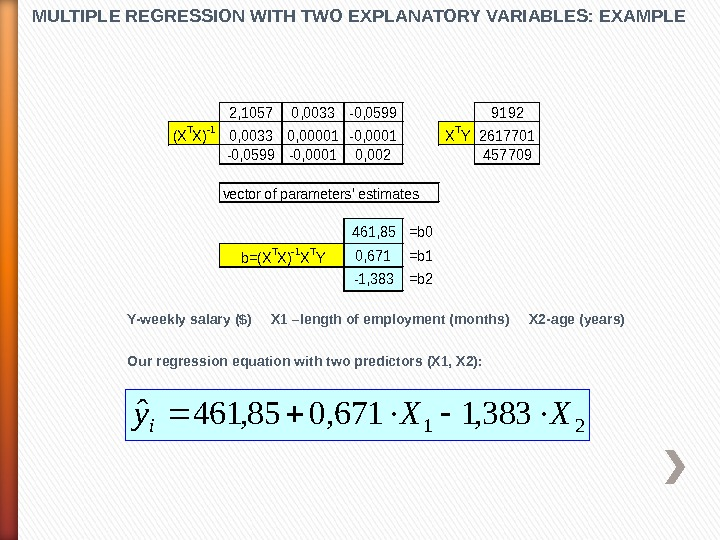MULTIPLE REGRESSION WITH TWO EXPLANATORY VARIABLES: EXAMPLE 2, 10570, 0033 -0, 05999192 (XTX)-10, 00330, 00001 -0, 0001 XTY 2617701 -0, 0599 -0, 00010, 002457709 461, 85=b 0 0, 671=b 1 -1, 383=b 2 vector of parameters’ estimates b=(XTX)-1 XTY Y-weekly salary (\$) X 1 –length of employment (months) X 2 -age (years) Our regression equation with two predictors (X 1, X 2): 21 383, 1671, 085, 461ˆ XXy i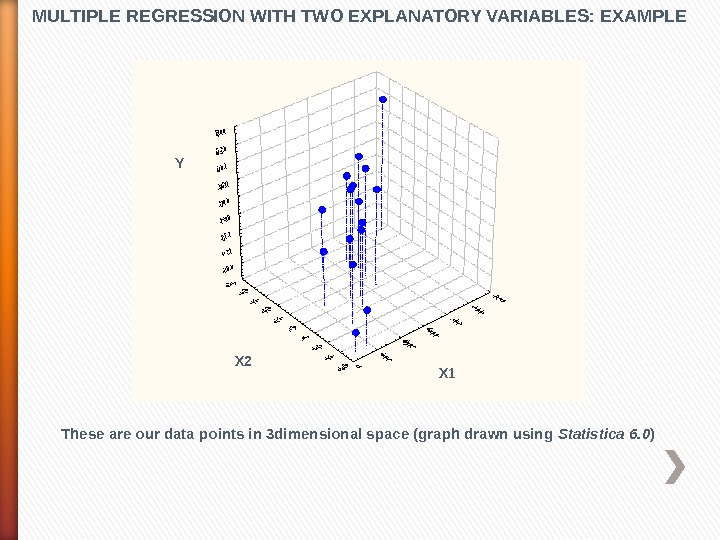MULTIPLE REGRESSION WITH TWO EXPLANATORY VARIABLES: EXAMPLE These are our data points in 3 dimensional space (graph drawn using Statistica 6. 0 )X 1 X 1 Y X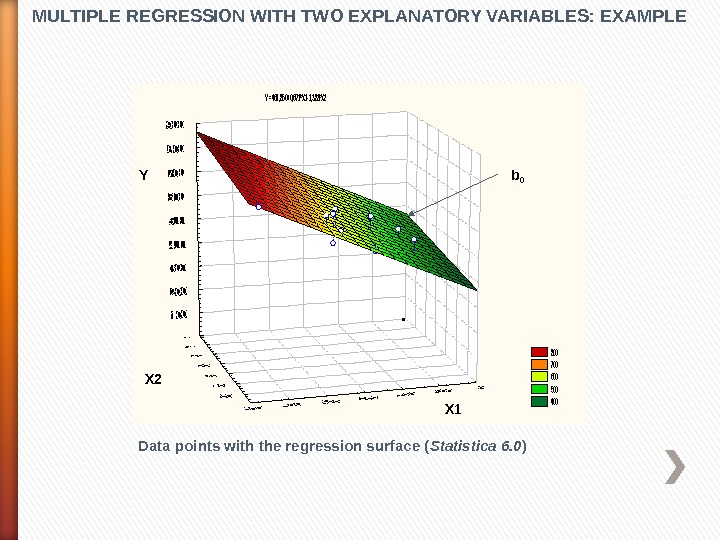MULTIPLE REGRESSION WITH TWO EXPLANATORY VARIABLES: EXAMPLEY =461, 850+0, 671*X 1 -1, 383*X 2 800 700 600 500 400 Data points with the regression surface ( Statistica 6. 0 )X 1 X 2 Y b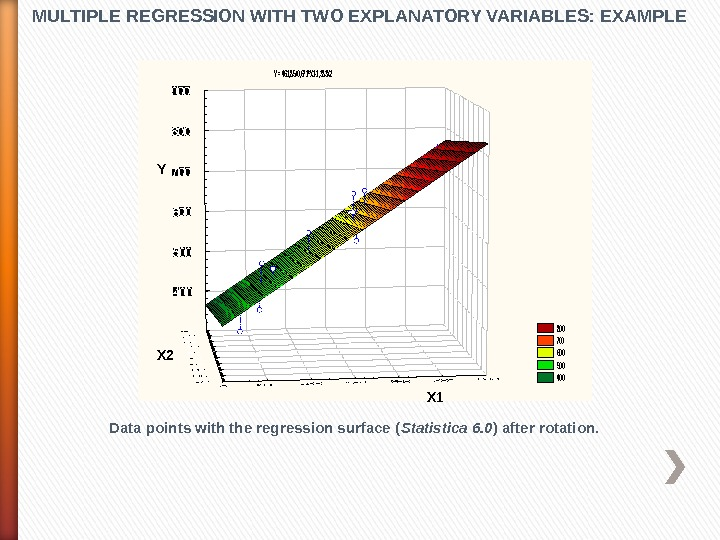MULTIPLE REGRESSION WITH TWO EXPLANATORY VARIABLES: EXAMPLE Data points with the regression surface ( Statistica 6. 0 ) after rotation. Y = 461, 85+0, 671*X 1 -1, 383 X 2 800 700 600 500 400 Y X 1 X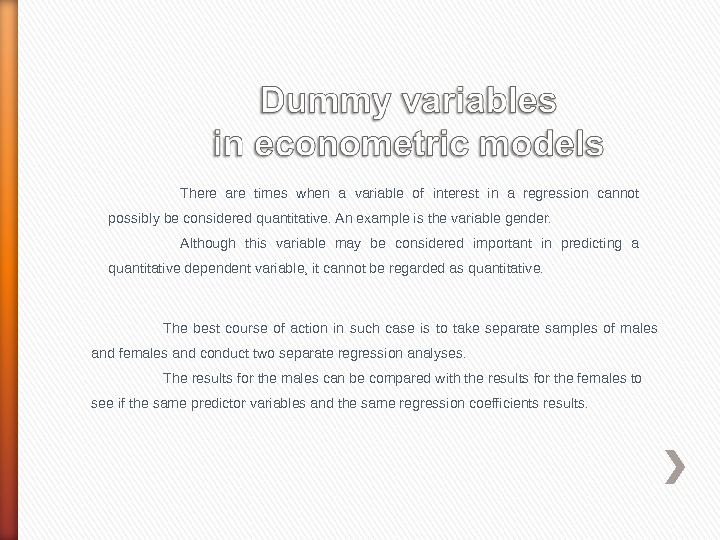There are times when a variable of interest in a regression cannot possibly be considered quantitative. An example is the variable gender. Although this variable may be considered important in predicting a quantitative dependent variable, it cannot be regarded as quantitative. The best course of action in such case is to take separate samples of males and females and conduct two separate regression analyses. The results for the males can be compared with the results for the females to see if the same predictor variables and the same regression coefficients results.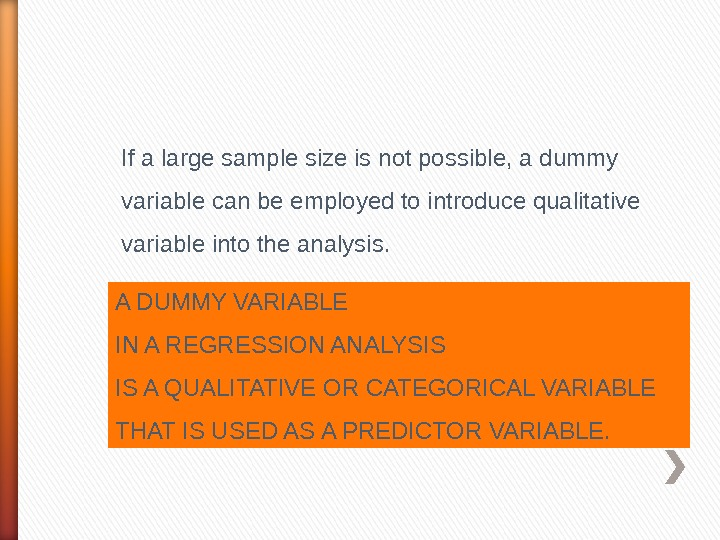If a large sample size is not possible, a dummy variable can be employed to introduce qualitative variable into the analysis. A DUMMY VARIABLE IN A REGRESSION ANALYSIS IS A QUALITATIVE OR CATEGORICAL VARIABLE THAT IS USED AS A PREDICTOR VARIABLE.For example, a male could be designated with the code 0 and the female could be coded as 1. Each person sampled could then be measured as either a 0 or a 1 for the variable gender, and this variable, along with the quantitative variables for the persons, could be entered into a multiple regression program and analyzed.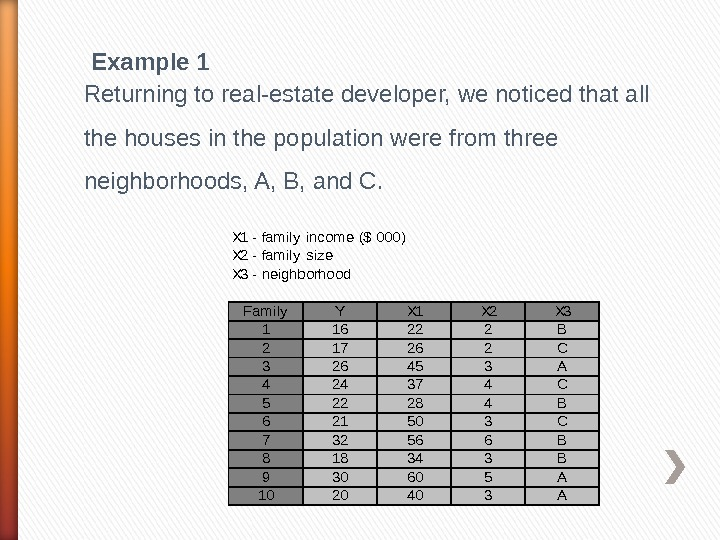Example 1 Returning to real-estate developer, we noticed that all the houses in the population were from three neighborhoods, A, B, and C. X 1 — family income (\$ 000) X 2 — family size X 3 — neighborhood Family. YX 1 X 2 X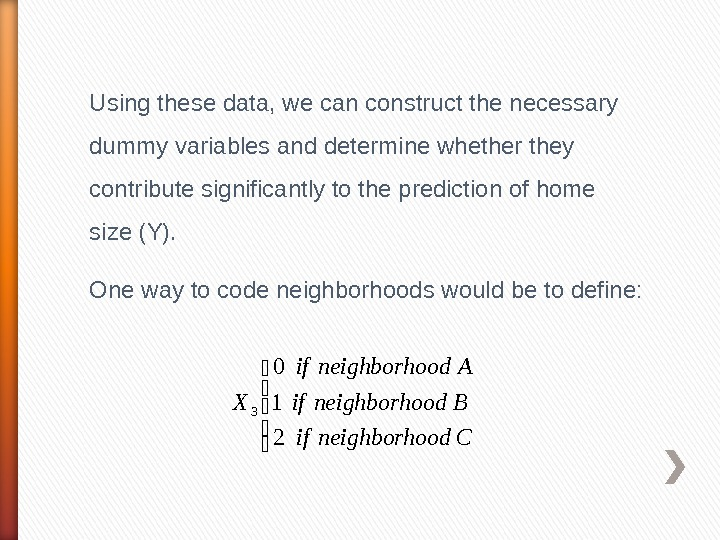Using these data, we can construct the necessary dummy variables and determine whether they contribute significantly to the prediction of home size (Y). One way to code neighborhoods would be to define: Codneighborhoif Bodneighborhoif Aodneighborhoif X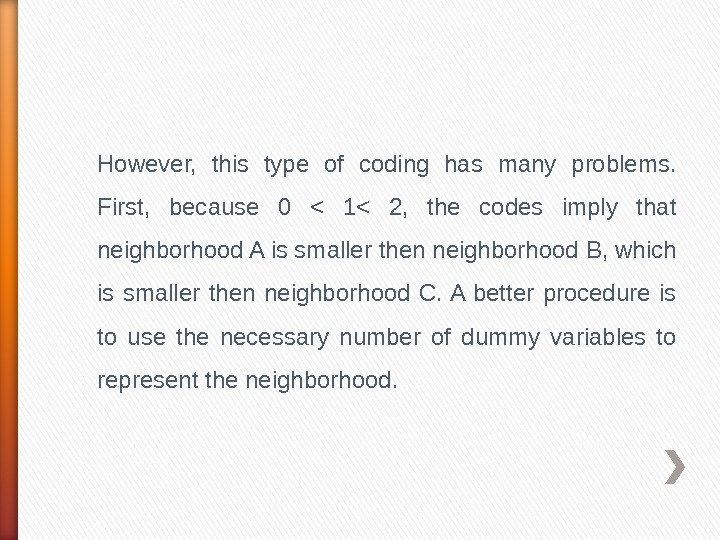However, this type of coding has many problems. First, because 0 < 1< 2, the codes imply that neighborhood A is smaller then neighborhood B, which is smaller then neighborhood C. A better procedure is to use the necessary number of dummy variables to represent the neighborhood.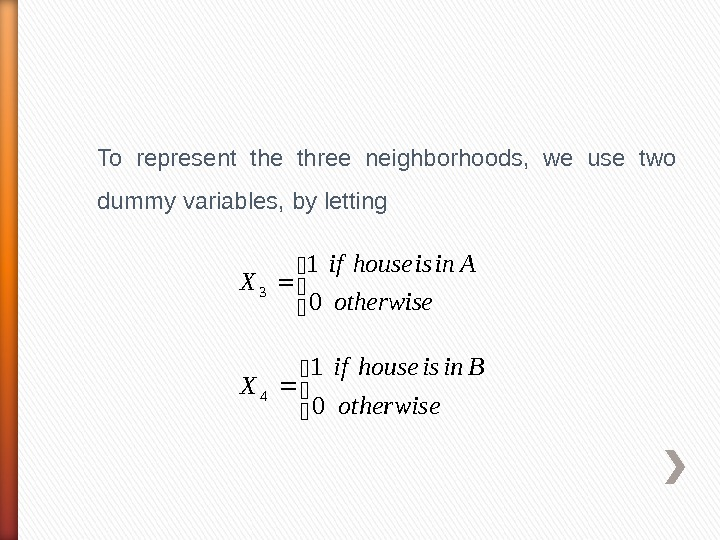To represent the three neighborhoods, we use two dummy variables, by letting otherwise Ainishouseif X 0 1 3 otherwise Binishouseif X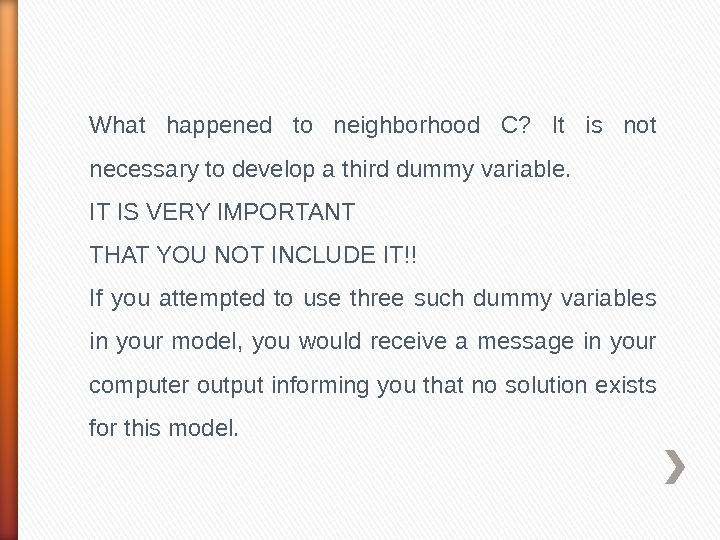What happened to neighborhood C? It is not necessary to develop a third dummy variable. IT IS VERY IMPORTANT THAT YOU NOT INCLUDE IT!! If you attempted to use three such dummy variables in your model, you would receive a message in your computer output informing you that no solution exists for this model.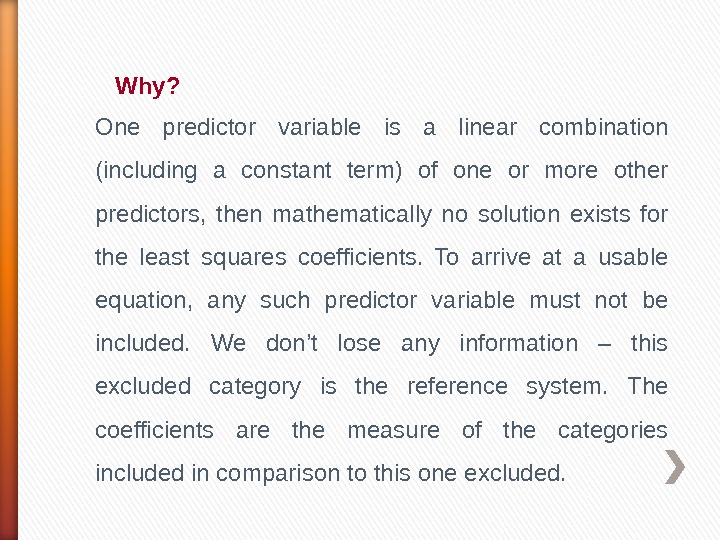Why? One predictor variable is a linear combination (including a constant term) of one or more other predictors, then mathematically no solution exists for the least squares coefficients. To arrive at a usable equation, any such predictor variable must not be included. We don’t lose any information – this excluded category is the reference system. The coefficients are the measure of the categories included in comparison to this one excluded.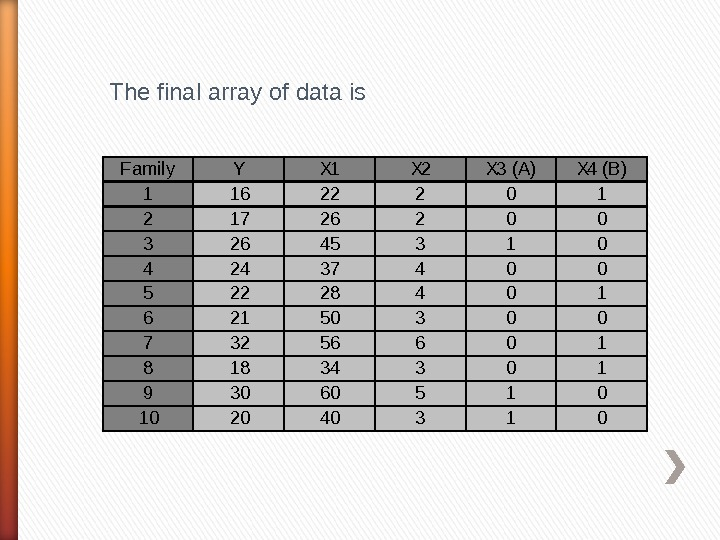Family. YX 1 X 2 X 3 (A)X 4 (B) 11622201 21726200 32645310 42437400 52228401 62150300 73256601 81834301 93060510 102040310 The final array of data is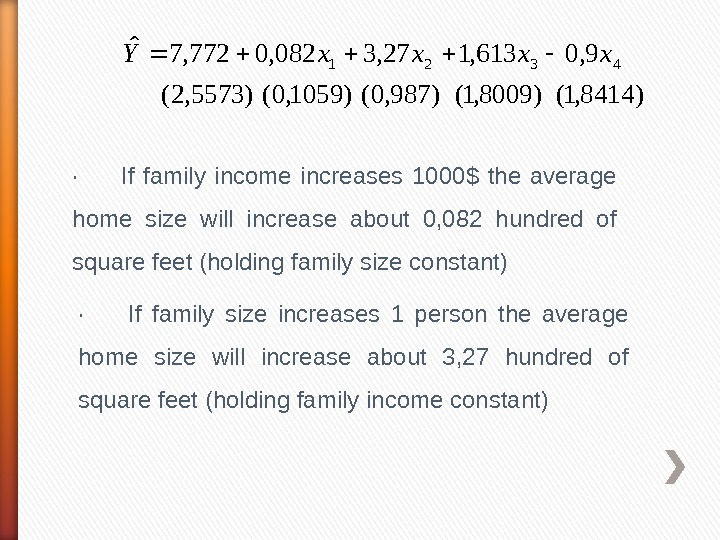)8414, 1()8009, 1()987, 0()1059, 0()5573, 2( 9, 0613, 127, 3082, 0772, 7ˆ 4321 xxxx. Y· If family income increases 1000\$ the average home size will increase about 0, 082 hundred of square feet (holding family size constant) · If family size increases 1 person the average home size will increase about 3, 27 hundred of square feet (holding family income constant)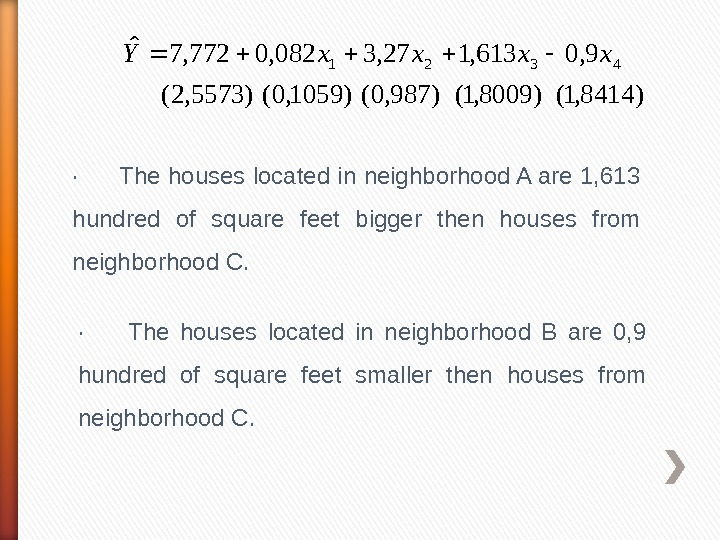)8414, 1()8009, 1()987, 0()1059, 0()5573, 2( 9, 0613, 127, 3082, 0772, 7ˆ 4321 xxxx. Y· The houses located in neighborhood A are 1, 613 hundred of square feet bigger then houses from neighborhood C. · The houses located in neighborhood B are 0, 9 hundred of square feet smaller then houses from neighborhood C.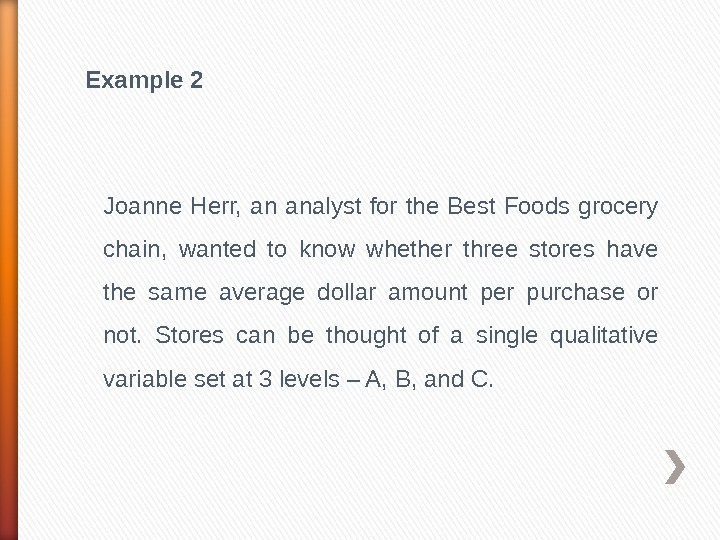Example 2 Joanne Herr, an analyst for the Best Foods grocery chain, wanted to know whether three stores have the same average dollar amount per purchase or not. Stores can be thought of a single qualitative variable set at 3 levels – A, B, and C.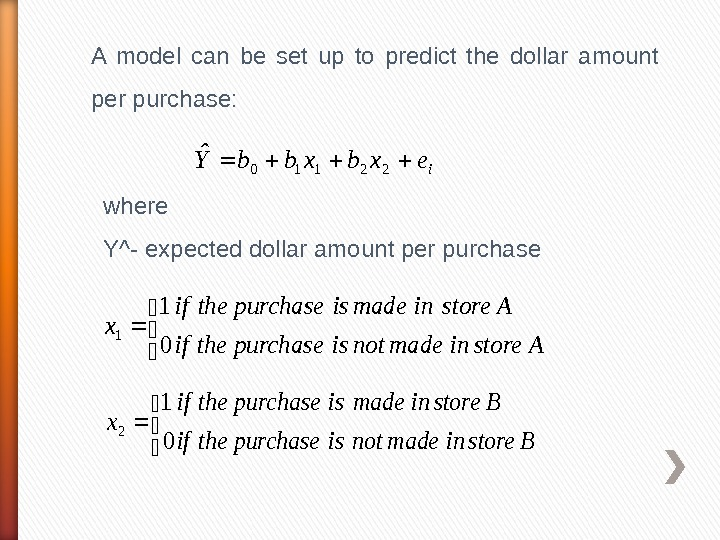A model can be set up to predict the dollar amount per purchase: iexbxbb. Y 22110 ˆ where Y^- expected dollar amount per purchase Astoreinmadenotispurchasetheif Astoreinmadeispurchasetheif x 0 1 1 Bstoreinmadenotispurchasetheif Bstoreinmadeispurchasetheif x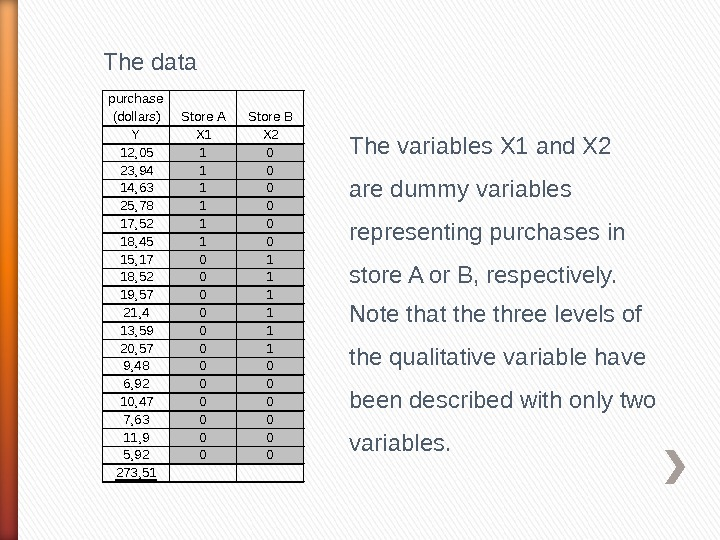The data purchase (dollars) Store A Store B Y X 1 X 2 12, 05 1 0 23, 94 1 0 14, 63 1 0 25, 78 1 0 17, 52 1 0 18, 45 1 0 15, 17 0 1 18, 52 0 1 19, 57 0 1 21, 4 0 1 13, 59 0 1 20, 57 0 1 9, 48 0 0 6, 92 0 0 10, 47 0 0 7, 63 0 0 11, 9 0 0 5, 92 0 0 273, 51 The variables X 1 and X 2 are dummy variables representing purchases in store A or B, respectively. Note that the three levels of the qualitative variable have been described with only two variables.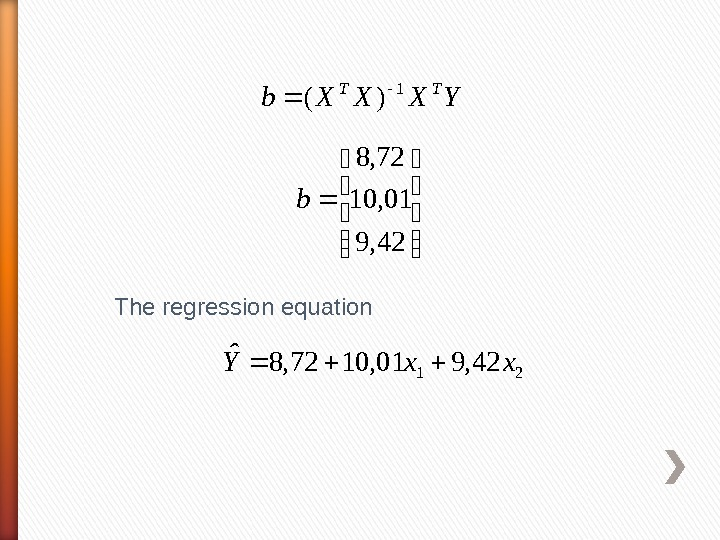The regression equation 2142, 901, 1072, 8ˆxx. Y YXXXb TT 1 )( 42, 9 01, 10 72, 8 b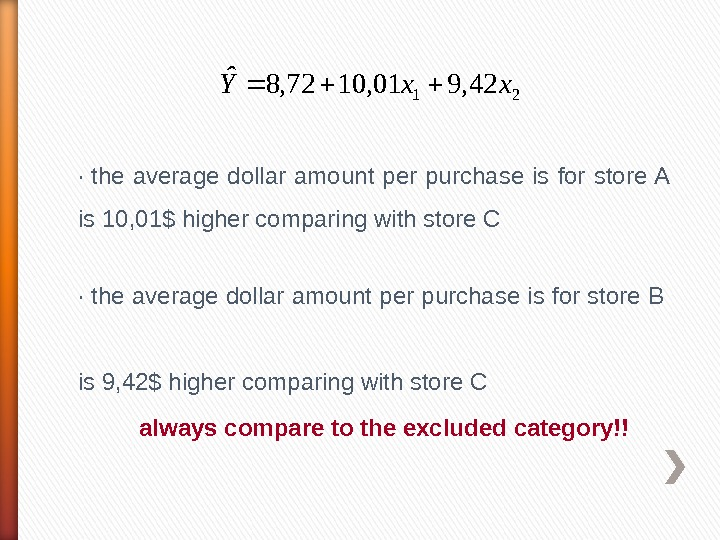2142, 901, 1072, 8ˆxx. Y· the average dollar amount per purchase is for store A is 10, 01\$ higher comparing with store C · the average dollar amount per purchase is for store B is 9, 42\$ higher comparing with store C always compare to the excluded category!!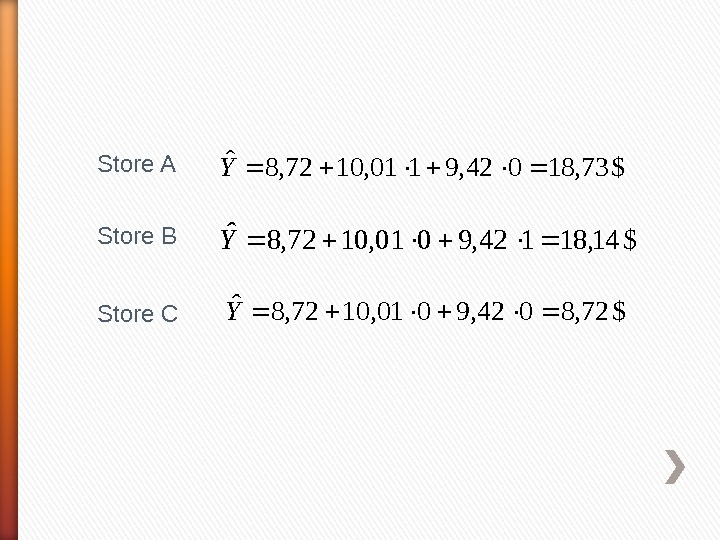Store A\$73, 18042, 9101, 1072, 8ˆY Store B Store C \$14, 18142, 9001, 1072, 8ˆY \$72, 8042, 9001, 1072, 8ˆY